# CS-241 - Foundation of Sequential Programs

## DISCLAIMER

These notes are far from complete and are quite porous compared to what’s been covered in class.
Copying code and diagrams off of powerpoints is hard in and of itself, but when the powerpoint slides start flying by, it becomes an impossible task.
Use these as a super rough outline of what we may have possibly covered in class, but do not use these as a primary set of study notes.

That said, the section regarding Formal Languages might be more complete.

Oh, one more thing!

I recently reimplemented the java-based VM used in CS 241 in C++, and I added a bunch of useful debugging features, including a step-by-step debugger.

It's called mips241 and you can get it over here!

Give it a whirl!

Yours,
- Daniel Prilik

# Instructor info

• Matt Crane
• Email: matt.crane@uwaterloo.ca
• Office Hours: DC2555F 3:00 - 4:00pm Tues / Thurs
• Troy Vasiga
• Email: troy.vasiga@uwaterloo.ca
• Office Hours: DC3112 12:00 - 1:30pm Tues / Thurs
• ISA: Max Bardakov
• Email: cs241@uwaterloo.ca

# Lecture 1

Oh boy! Here we go again!

## Bookeeping

The course website is www.student.cs.uwaterloo.ca/~cs241/

• Syllabus
• Announcements
• Everyhing else!

• Start early!
• Don’t fall behind!
• In total, there are 10 assignments, each with ~9 subparts, so ~100 things to submit

The course uses our favorite program: Marmoset!

• This time around though, there are Release Tokens, that give you 3 attempts per 12h to test your programs

Marking breakdown:

• 25% Assignments - Due Thursdays at 7:00pm
• 25% Midterm - Wed Oct. 26th from 7:00-8:50pm
• 50% Final (must pass) - 2.5h sometime in December

## Idyllic Intro

So what is the purpose of this course:

• To learn
• To Meta-think
• To write a program, that reads a program, that outputs a program.
• Inception. BWUHHH

By the end of this course, we will know what really happens when you compile and run a program, and there will be little to no mystery about what happens when you run a program.

## Technical Intro

In this course, all a computer is a CPU and RAM.

A CPU Controls and Manipulates the Data, and the RAM is just a bunch of addressed “boxes” that store Data.

## Binary Data

All data is at a basic level is a sequence of 1s and 0s, or Bits

There are many interpretations for what a specific sequence of bits means (i.e: numbers, ascii, etc…), and there is no one “correct” interpretation.

## Machine Language

There are certain sequences of bits that do in-fact have one specific meaning, or at least they do when it comes to how the machine interprets them. Depending on the Achitecture of the machine (i.e: Intel/Motorola, 32/64bit, etc…) there may be different bit sequences that mean the same things.

These codes are great for machines, super easy, just 0 = off, 1 = off, but they SUCKS for humans.

## Assembly

Assembly on the other hand, sucks less for Humans.

It is a simple, textual representation of machine language. It is more human readable, and makes development less, shall we say, absolutely terrible.

A big plus of assembly is that is it pretty easy to translate Assembly to Machine Code. Assembly is just an Abstraction.

## Assembler

The Assembler is the program that preforms the translation from Assembly to Machine Code.

## Back to the Basics: Bits

What is a Bit exactly?

Well, it is just a value that can have 2 states, either 1, or 0. That’s it.

The nice thing is that a sequence of bits can describle a lot of different value.

• 2 bits can have 4 arrangements
• 3 bits can have 8 arrangements
• n bits can have 2^n arrangements!

We can use Bits to describle certain useful “types”:

### Integers

#### Unsigned integers

Each sequence is just a direct representation of a number in base 2.

#### Two’s Compliment

What if we want Negative Numbers? Well, then we have to use a more complicated code, called 2s compliemnt.

To get 2s compliemnt, we

1. write the absolute value of a number in banary
2. Negate the bits (to get 1s compliment)

So, With 3 bits, we can have the following interpretations for integers…

Binary Decimal 2’s compliment
000 0 0
001 1 1
010 2 2
011 3 3
100 4 -4
101 5 -3
110 6 -2
111 7 -1

** Notice that in 2s compliment, the left-most bit tells us if it is negative or not…

To hammer in this point, consider 1010.
What does it mean?
Well, nothing really, not until we know what type is should represent.
It is 10 if it’s an unsigned number, but it’s -6 in 2s compliment

#### Characters

Typically represented with 8 Bits

Eg: 00001010 is \n in ASCII (use the linux command man ascii for a full table)

In general, binary SUCKS to work with. Hexadecimal sucks less.

Essentially, we can represent numbers with 16 symbols, instead of just 1 and 0

We use the digits 0-9, A, B, C, D, E, and F.

A nice property of Hexadecimal is that it is easy to convert into Binary, and vice versa.
You just split the binary number into chunks of 4 bits, and then write the digit associated with each chunk.

Eg: 1010 1111 1001 1001 = xAF99

# Lecture 2

## Grouping of Bits

The most common grouping of bits is in a byte, or a group of 8 bits

There are 256 possible values that can be encoded by 8 bits. What to encode however, is up to interpretation (see above)

## Files

Files are just long sequences of bytes, and it is up to a program to determine what the byte sequences actually mean.

The command cat for example, will interpret any file thrown at it as Text, and print out each byte as a ASCII char.

The command xxd on the other hand, will print the file’s bits as hexadecimal values (alongside an ascii interpretation of the bits)

So, for example, if there is a file hasY with string Y\n, then the output of xxd would be something like:

#Addr     #Hex   #Ascii
00000000: 590a   Y.


59 is the hex code for Y in ascii, and 0a is the hex code for the Newline Char in ascii

## Words

A Word is a grouping a bits that a specific CPU “likes” (i.e: can easily do math on, execute ops, etc…)

Words can be any number of bits… from 8 bit in the 80s, all the way to 64 bit nowadays.

NOTE: a CPU’s specific word-size will limit the total ammount of memory a CPU can ever see! For example, 32 bit CPUs could only address ~3.5gigs of memory, so until the switch to 64 bit CPUS, no matter how much RAM you shoved in a PC, if the OS was 32bit, it couldn’t see more than 3.5gigs

## Our Machine

The machine we are using in cs241 is a Stored Program Computer

What this means is that the CPU is connected to the Memory (via a “mem bus”) not just to use the memory as a place to store data, but also as a place to store actual program code.

To run a program, the CPU calls the memory for an instruction, and the memory returns a string of bits that (should) correspond to a instruction that the CPU can execute.

## CPU

CPUs are not actually just magic boxes. They have things within them that make them work…

There are several places to Store things

• Program Counter (PC)- Where in memory to load new instructions from
• Instruction Register (IR)- Chunk of memory that holds the instruction to run
• Registers 0-31 ($0 ->$31) - “boxes” that can store words of information (think superfast ram)

And there are other places that Do things

• Control Unit - reads the PC, retrieves the instruction from memory, puts that instruction in the IR, and then executes the instruction
• Arithmetic Logic Unit (ALU) - Can do maths on given bits. Things like adding, subtracting, AND / OR / NOTs, etc…

## Memory (RAM)

RAM is really just a giant block of “boxes” that can store bytes, starting from 0 to denote the 1st byte, 4 to denote the 5th byte, etc…

On a MIPS machine, words are 4 bytes, and as such, when addressing the RAM to ask for instructions, we must ask for words, so we address RAM in increments of 4.

## MIPS

MIPS is a set of instructions that our processor understands.

In this course, there are 18 different 32-bit (4 byte) instructions encoded in 2 basic instruction formats.

MIPS assembler is the Assembly Language that translates human-readable instructions into the actual 32-bit / 1 word instructions that the CPU can read.

For example:

• add $1,$2, $3 are MIPS instructions that correspond to… • 0000 0000 0100 0011 0000 1000 0010 0000 when converted to Binary, or • 0x00430820 when written in Hex That binary actually means the following… 000000 ; Call the ALU 00010 ;$2
00011       ; $3 00001 ;$1


A full list of MIPS instructions can be found here: https://www.student.cs.uwaterloo.ca/~cs241/mips/mipsref.pdf

The instruction lw loads a word from memory, and sw stores a word to memory

Eg: lw $7, 0($3) will load the value at MEM[$3] into register$7
Eg: sw $8, 4($3) will load the value from $8 to MEM[$3+4] (that +4 is needed since we store Words in memory, and Words are 4 bytes each)

## Main Machine Cycle

Every single clock cycle, the following happens:

1) Fetch a word from RAM whose address is in the PC (the PC is initialized to 0 on power-on)
2) Place that word in the IR
3) Increment the PC by 4
4) Decode and Execute the instruction that is in the IR

# Lecture 3

## Special Registers

Register Description
$0 Constant value 0 $29 and $30 Stack (see below…) $31 The Return Address (RA)

There is a 1 to 1 correspondance b/w Assembly Language and Machine Language. It translates human-friendlier mnemonic instructions like add $3,$1, $2 to their related bit form. The Assembler is the program that does this automatic translation. For some example of Assembly Code and the Machine Language equivilents, see the cs241 website. # Lecture 4 ## Labels Labels identify an address. ; some stuff loop: add ... ; more instructions... bne$2, $0, loop ;using label X: Y: ; can have 2 labels on the same line  ## Storing and Restoring Registers What happens if we call a subroutine where it uses some registers, that you need! We only have$32 registers after all!

Consider this example below

; Example 6a: Calling a procedure
sw $31, −4($30)        ; save $31 on stack lis$31
.word 4
sub $30,$30, $31 lis$1                 ; call sumOneToN(13)
.word sumOneToN
lis $2 .word 13 jalr$1
lis $31 ; restore$31 from stack
.word 4
add $30,$30, $31 lw$31, −4($30) jr$31                 ; return to OS

;------------------------

; Example 6b: A procedure
; sumOneToN: sum the integers from 1 to N
; input: $2 is N ; output:$3 is the sum

sumOneToN:
sw $1, −4($30)         ; save $1 on stack sw$2, −8($30) ; save$2 on stack
lis $1 .word 8 sub$30, $30,$1
add $3,$0, $0 ; clear$3
beginLoop:
add $3,$3, $2 ; add$2 to $3 lis$1                 ; decrement $2 .word −1 add$2, $2,$1
bne $2,$0, beginLoop
lis $1 .word 8 add$30, $30,$1
lw $1, −4($30)         ; restore $1 from stack lw$2, −8($30) ; restore$2 from stack
jr $31 ; return from sumOneToN  In general, Stack usage rules are: sw sw ... sub$30, $30, 4.n [body] add$30, $30, 4.n lw lw jr$31


## Recursion

Here is an example of recursion

; Save $31 on the stack sw$31, -4($30) lis$31
.word 4
sub $30,$30, $31 ; Call recSum(13) lis$4
.word recSum
lis $1 .word 13 jalr$4

; Restore $31 from the stack lis$31
.word 4
add $30,$30, $31 lw$31, -4($30) ; Return to OS jr$31

;-----------------------

; recursively sum up the integers from 1 to N
; assume that input (N) is in $1 ; output is returned in$3
recSum:
; Save registers on the stack
sw $1, -4($30)
sw $2, -8($30)
sw $4, -12($30)
sw $31, -16($30)
lis $4 .word 16 sub$30, $30,$4
; Initialize sum so far
add $3,$0, $0 ; Check to see if we are in the base case (N=0) beq$1, $0, done ; Otherwise, we must compute the sum of the current N ; and the sum of the rest ; keep a copy of the current value of N add$2, $1,$0
; put N-1 into register $1 lis$4
.word 1
sub $1,$1, $4 ; get ready to call routine (i.e., ourself) lis$4
.word recSum
jalr $4 ; add the value we got back to the current value of N (in$2)
add $3,$3, $2 done: ; restore registers lis$4
.word 16
add $30,$30, $4 lw$1, -4($30) lw$2, -8($30) lw$4, -12($30) lw$31, -16($30) jr$31


In general, the template for recursion in MIPS is roughly:

• Save registers ($31 in particular) • Check base case • Recursive case: • Compute next value • Call self • Compute return value • Restore registers # Lectures 5 ## Assembler Stages First pass = make an intermediate representation Second pass = make actual ML code ## Syntax and Semantics Syntax = form / structure (col 2 of MIPS ref sheet) Asm lang syntax goes in, ML lang syntax comes out Semantics = “meaning” (col 3 of MIPS ref sheet) What do instructions actually “do” Location and Labels (col 4 of MIPS ref sheet) Location = “where I am” Label = “where I want to be” ## First Pass - Analysis Must generate 1) an intermediate representation 2) a symbol table Tokenization (not neccesary, but probably the best intermediate representation • A Tokenizer is a program that • takes an Input of ASCII chars • Outputs a sequence of Tokens • e.g: add$3, $1,$7 => <ADD><REG><COMMA><REG><COMMA><REG>

### Intermediate Representation (IR)

There is no “hard and fast” rules for what a IR can be.
It can be anywhere from

• Just the raw ASCII minus comments
• A series of tekens
• Perliminary binary translation
• Or really anywhere inbetween

### Symbol Table (ST)

Stores the address and the name of each label definition

The following code has the following Symbol table

0x00 misery: beq $0,$7, happy
...
0x0C         beq $7,$12, misery
...
0x1C happy:  add $3,$3, $3  name address misery 0x00 happy 0x1c ## Second Pass - Synthesis Takes the IR and ST, and combines them into valid machine code ## Example: Encoding a beq instruction Given 0x0C beq$7, $12, misery, and that misery is at 0x00 from the MIPS reference sheet, we know beq has format 0001 00ss ssst tttt iiii iiii iiii iiii, where i is an Offset from the current PC! Thus, we must encode those i‘s to have a value of -16, to move the PC back to 16 ## Advice for making an assembler Look for patterns! For example: ADD and SUB have instructions that are almost the same! They only differ by 1 bit! There are plenty of these, so don’t forget to write DRY code ## Efficieny of the Symbol Table For large programs, there will be a huge symbol table, and there must be a way to have quick and efficient access to it. Symbol Tables are just (key, val) pairs, with the key being the label string, and the value being an integer representing the address of the label So, TL;DR, use a Hash Map / Dictionary • Supports quick insert (Pass 1) • Supports quick remove (not that we will be doing any removes) • Supports quick finds (Pass 2 AND Pass 1 - Check for same label, 2 addr) ## Error Checking in Pass 1 Check for Duplicate Label Definitions (i.e: same label for 2 different addresses) Check for Label Syntax • Must start with an alphabetic char (so 123fred: is bad,$54: is bad)
• Must have only alphanumeric chars

Check basic Syntax

• Eg: asddsa fgdfg ,,; is invalid

## Error Checking in Pass 2

Consider beq $3,$4, notALabel

We can’t jsut throw this instruction out in Pass 1, as notALabel might be defined later. Only in Pass 2 can we throw a “label undefined” error
This is a Semantic Error

Consider lw $3 4($30)

This will throw a Syntax error

Consider lw $3, 4($30
Consider lw lw $3, 4($30)

These will all throw Syntax error

Consider lv $3, 4($30)

This will thow either a “invalid instruction” error, or a “Syntax error”

Consider lw $3, 4($32)

This will throw a Semantic error, since register $32 doesn’t exist Consider lw$3, 44444444444444($30) This will throw a Semantic error, since that number is out of the 16 bit bound, and can’t be encoded in the instruction Consider $3, 5($30) This might throw a Semantic error, since 5 is not a multiple of 4 (but i might not, if the multiplication between$30 and 5 is actuall a multiple of 4)

## Error Checking Philosophy

### Accept valid instructions, reject everything else.

Easier to check validity of instruction, than the invalidity of the instructions.

## A note on what the Assembler will output

The assembeler we write will output literal bits, NOT ASCII chars!!

That means that the assembler will make heavy use of the bitwise operators, like &, |, <<, >>, etc…

Output is still to STDOUT, but use putchar

On the Assignment 3/4 page, you can download asm.c / asm.cc / asm.rkt, that will provide a significant ammount of helpful boilerplate code to jumpstart your assembler development.

## How to Write an Assembler

Slowly.

With a lot of tests.
Like, a LOT of tests.
Like a SHITTON of tests.
Like an UNREASONABLE AMMOUT of tests.

## Correctness

• Read the spec carefully, or, RTFM
• Think reasonably, and unreasonably
• Remember memory: O(n)
• Running times DO matter: O(n)
• Dont’t use Marmoset as your only testing tool!

# Lecture 6

a) delegates RAM to programs,
b) copies those programs to that point in ram,
c) executes them.

In pseudocode, the loader does the following:

loop
decide what program to run
figure out the length of the program (n)
find n words of storage at some address (a)
read program into memory at a
set up program (e.g: mipstwoints)
put a into a register (say $19) jalr$19
end loop


## Chickens and Eggs

To get the size of the program we want to load, we actually have to store the size of our program with our program (this is because of the chicken and egg problem of having to load a program into RAM to find it’s size, but we need it’s size to load it into RAM)

The Firmware / BIOS

It’s turtles all the way down.

(this is called Bootstrapping)

### NOTE

I missed the next bit of the lecture, so get ready for some pretty disjointed notes.

## MERL Format

Header cookie (1 word: beq $0,$0, 2 (0x10000002))
Length of the .merl file (1 word)
Length of program + header (1 word)
Program code (A3 + A4)
Notes of what to change (Relocation entries: .word 1 .word <loc>)
- i.e: which registers link to other registers that would have to have a mem-offset added to them

See full description on the CS241 website

## A MERL Assembler (for A5)

• Pass 1 changes
• Start counting at 0xc to account for the 3 word MERL header
• If you see a .word <label> instruction, write down it’s location in the relocation table
• Pass 2 changes
• Output the header (the coockie, the size of the .merl file, the size of the program + header)
• Output notes:
• Relocation table entries

read header
a = findFreeRAM(codeLength)
for each instruction:
MEM[a + i] = instruction
for each relocation entry
MEM[a + location] += a
place a into $19 jalr$19


# Lecture 7

## MERL file for example code

Code

A: .word B
B: .word 7
C: .word 0xA
D: .word C


Symbol Table

A 0x0C
B 0x10
C 0x14
D 0x18

Relocation Table

Relocations
0x0C
0x18

The Merl File:

; Header
0x10000002
0x0000002C
0x0000001C

; Program Code
0x00000010
0x00000007
0x0000000A
0x00000014

; Relocation Entries
0x00000001
0x0000000C
0x00000001
0x00000018


Linkers take distinct programs, and smush them together into one package.
This is useful to create reusable components of code, and allow other code to to them.

## Missed a lot about ESD and ESRs

concatenate the (merl) programs
construct the ESD
use ESR
Relocate (internally)


# Lecture 8

• Assemble and combine all code into one large, self contained, executable

• Done at execution time
• Essentially, you call a “system subroutine” with the argument being a string of the subroutine you actually want to find, and the system will then redirect you to the code you want.
• Results in smaller file-sizes
• Multiple programs reuse the same subroutines

That’s all for MIPS btw

# An Introduction to Formal Languages

## Motivation

• Precision of specification and recognition
• Importance of this: every assignment past A5 will use these concepts
• Benefits of theory:
• Means ofcommunication
• Determine the power and limits of communication (what we can / can’t express)
• Guides the design and structure of a compiler

## Terminology

• Rooted in set theory {}
• Alphabet: a finite set of symbols
Σ1={a,b,c}$\Sigma_1 = \{a, b, c\}$
Σ2={Δ,cat,blue,123}$\Sigma_2 = \{\Delta, cat, blue, 123\}$
• Word (aka strin, sentance): a finite sequence of symbols from the alphabet

• Words over Σ1:W1=baab  W2=aaa  W3=ϵ(orλ)$\Sigma_1: W_1=baab\ \ W_2 = aaa\ \ W_3=\epsilon(or \lambda)$
• Note that no symbols is totally okay.
• Language: a set of words

• Languages over Σ1:$\Sigma_1:$
• L1={a,aa,aab,baa}$L_1 = \{a,aa,aab,baa\}$
• L2={a,aa,aaa,aaaa,...}$L_2 = \{a,aa,aaa,aaaa,...\}$
• L3={}$L_3 = \{\}$
• L4={ϵ}$L_4 = \{\epsilon\}$
• |W|$|W|$: the size of W$W$ (Where W$W$ is a word or language)
• |L1|$|L_1|$ = 4
• |L2|$|L_2|$ = infinite
• |L3|$|L_3|$ = 0
• |L4|$|L_4|$ = 1
• |W1| (baab)$|W_1|\ (baab)$ = 4

## Use of Formal Languages Specification

A statement of what a language is should be

• precise (no ambiguity)
• easy to express
• Automatable

We can use these specifications to Determine if a word is in a language

• Formally: given language L$L$ and a word w$w$, recognition answers the question: “is wL$is\ w \in L$
• Techincally, this is just a Yes or No question, but in practice, we would like some info about what went wrong
• Eg: if NO: line # and type of error, if YES: certificate of correctness

## WLP4

Let’s formally specify WLP4 “WooL P Four” = “Waterloo, Language, Plus, Pointers, Plus, Procedures”

• Alphabet: WLP4 tokens
• Word: A WLP4 program
• Language: Set of calid WLP4 programs

There is a full language specification the main course web-page: https://www.student.cs.uwaterloo.ca/~cs241/wlp4/WLP4.html

## Language Classes

Language classes are sets of languages that may share common characteristics

• Chomsky Hierarchy for Languages (n+1 encompases n):
• Finite Languages (fixed number of words)
• Regular Languages (a, aa, aaa, aaa…)
• Context-free Languages (explained later)
• Context-sensitive Languages (explained later)

As we move down the chompsky hierarchy, we move up in power of expressiveness, but also complexity

## Use of Formal Languages - Organization of Compilation

• Lexical analysis - Regular Languages
• Syntaxtic analysis - Context Free Langauges
• Context-sensitive analysis (semantic analysis) - Context Sensitive Languages
• Synthesis (code generation) - Context Sensitive Languages

## Which Language Level is it?

• Let Σ={ASCII characters}{CR}$\Sigma = \{ASCII\ characters\} - \{CR\}$
• L1={$L_1 = \{$0, 1,$1,$2, … 31}$31\}$
• Finite Language
• L2=$L_2 =$ valid labels in MIPS
• Regular Language
• L3={0,4,4,8,8,...}=$L_3 = \{0,4,-4,8,-8,...\} =$ valid load word (lw) offsets
• Finite Language (offsets have bounds)
• L4=$L_4 =$ valid line of assembly language for A3P2 (.word <int> or .word <hexint>)
• Finite Language (ints/hexints have bounds)
• L5=$L_5 =$ valid line of assembly language for A3P3 (+.word <label>)
• Context Sensitive (validity depends on if label is defined)

# Lecture 9

## Specifying Regular Languages

There are several basic building blocks that we use to specify Regular Languages:

### Finite languages

You can always build off an existing finite language

### Union

Formally Defined: T1T2={x | xT1 or xT2}$T_1 \cup T_2 = \{x\ |\ x \in T_1\ or\ x \in T_2\}$
Simply: Make a new set containing all the elements of both sets (with each element appearing only once)

### Concatenation

Formally Defined: T1T2={xy | xT1and yT2}$T_1 \cdot T_2 = \{xy\ |\ x \in T_1 and\ y \in T_2\}$
Example:
T1$T_1$= {dog, cat}
T2$T_2$= {ϵ$\epsilon$, fish}
T1T2$T_1 \cdot T_2$ = {dogfish, catfish, dog, cat}

T1$T_1$ = {dog, cat}
T2$T_2$ = {}
T1T2$T_1 \cdot T_2$ = {}

### Repetition

Formally Defined Recursively (Kleene closure):
T={ϵ}{xy | xT and yT}$T* = \{\epsilon\} \cup \{xy\ |\ x \in T*\ and\ y \in T\}$
Alternate Definition (Iterative):
T0={ϵ}, T1=T, T2=TT1={xy | xTand yT1}$T^0 = \{\epsilon\},\ T^1 = T,\ T^2 = T \cdot T^1 = \{xy\ |\ x \in T and\ y \in T^1\}$
(In general): Tk=TTk1$T^k = T \cdot T^{k-1}$

Examples:
T=T1=$T = T^1 =$ {dog, cat}
T$T*$ = {ϵ$\epsilon$, dog, cat, catdog, dogcat, dogdog, catcat, dogdogcat, …}

## DFAs (Deterministic Finite Automata)

Regular Languages can be recognized by Finite Automota
We begin with deterministic finite state automata (DFAs)

DFAs must have the following:

• States (at least one)
• Start State (ONLY 1)
• Final State(s) (MUST HAVE MIN 1)
• after reading all input, if I am in a final state => accept
• Transitions (optional - eg: no transitions with 1 state)
• if in state A AND read input xΣ$x \in \Sigma$ => move to state B

A DFA for some MIPS instruction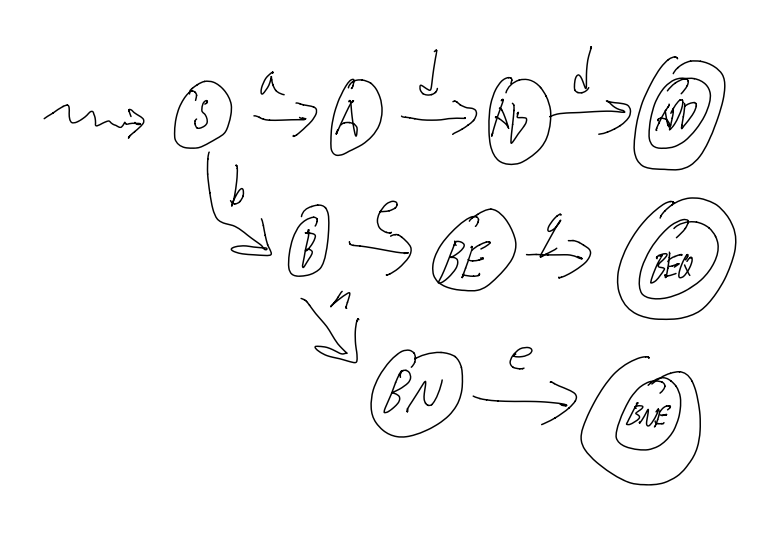A DFA for a label (i.e: starts with letter, then any numberletter or number)Images courtesy of Stephen Melinyshyn

• Ability to trace
• can draw a picture (only ever in one state)
• Transitions out of states are unique and deterministic
• Errors -> implicit error state (if input is not specified in a transition of a state, then that is an error)

## Formal Definition of DFAs

• A DFA is a 5-tuple (Σ,Q,q0,A,δ)$(\Sigma, Q, q_0, A, \delta)$
• Finite Alphabet Σ$\Sigma$
• Finite set of States Q$Q$
• Start State q0Q$q_0 \in Q$
• Set of final/accepting states AQ$A \subseteq Q$
• Transition function δ:Q x ΣQ$\delta : Q\ x\ \Sigma \to Q$

## DFA Interpreter Algorithm

Input: A word w=w1w2...wn$w = w_1w_2...w_n$ where each wiΣ$w_i \in \Sigma$
Output: true if accepter, false if rejected

state = q0
for i in 1..n:
state = delta(state, wi)
return (state in A)


## Implementing DFAs

We need to implement the transition function somehow… but how?

Option 1: if statements?

if state == state1:
if input == a: ...
else if input == b: ...
...
else if state == state2:
if input == a: ...
...
...


NO. THIS IS A TERRIBLE IDEA.

Option 2: Dictionary: key = (state, input), value = state

## Where are DFAs used?

• Every computer ever
• a CPU is essentially just a DFA

# Lecture 10

## Non-deterministic Finite Automa (NFAs)

L = {bba, baa, bbaa, bbbaa, bbbbbaa, …} (wich is either 2 b’s followed by an a, or 1 or mote b’s bollowed by 2 a’s)

If we try to derive this using a DFA, it is pretty unweildy, and grows pretty large. Moreover, it is hard to see what the DFA recognises at a glance… TL;DR: It works, but it’s ugly.

On the other hand, if we do this with an NFA, it becomes a lot clearer.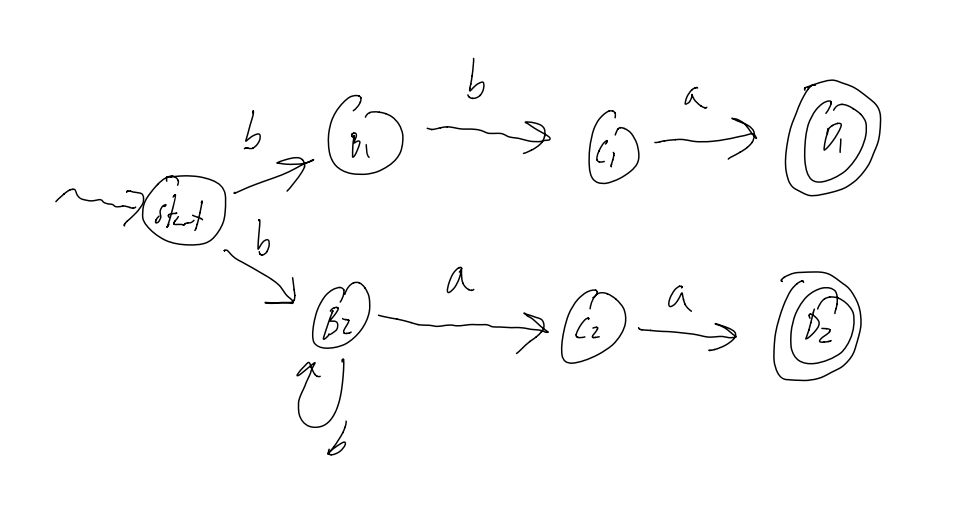NFAs can be in 2 different states at the same time!
NFAs allow multiple transitions out of one state on the same input

## NFA Definition

It’s the same as DFA with the following change: T:Q x Σ2Q$T:Q\ x\ \Sigma \rightarrow 2^Q$
In english: We can be in a Set of States, and this T is a relation instead of a function

2q is the Powerset of Q

if Q = {a,b,c} then 2q = { {},{a},{b},{c},{ab},{ac},{bc},{abc} }

Note: |2Q| = 2|Q|

## NFA interpreter

Input: a Word w=w1w2...wn$w = w_1w_2...w_n$
Output: True$True$ if accepted, False$False$ if rejected

Pseudocode:

states = {q_0}
for i in 1..n:
states' = {s' | s' in T(s,w_i) for all s in states}
states = states'
return (states) instersected with (Accepted States) =/= {}


Essentially, we have to track an array of possible states we are in. That’s all.

## Implementing an NFA interpreter

With C++, we are lucky enough that STL provides us with methods like:
- Intersection
- Union
- Membership
- Iteration

BUT these are NOT constant time operations.

Luckilly, we can improve preformance by using a Bit Vector
i.e: Say we have 8 states, and we have a byte like 10101100, that means we are currently in sates 3,4,6,8

Using bit vectors lets us use bytewise operations to do intersection, union, etc… but we have to deal with bitshifting :D

## Differences b/w NFAs and DFAs

NFAs can be smaller than DFAs for the same language
NFAs can be in multiple states at the same time
DFAs are easier to implement due to one variable (the current variable)

NOTE: The two are equivalent in the languages they recognize.
i.e: you can always write a DFA from an NFA and you will have to on the midterm
Also, all DFAs are valid NFAs.

## Killer app for Finite Automa

Scanner = tokenization, and indeed, if you look at the source code given for the asm assignment, you can see an implementation of a tokenizer thant uses DFAs.

## ϵ$\epsilon$-NFAs

Allow transitions between states on “no input”
Can be used as “glue” for joining machines together

Example: L = {card, cab, calf}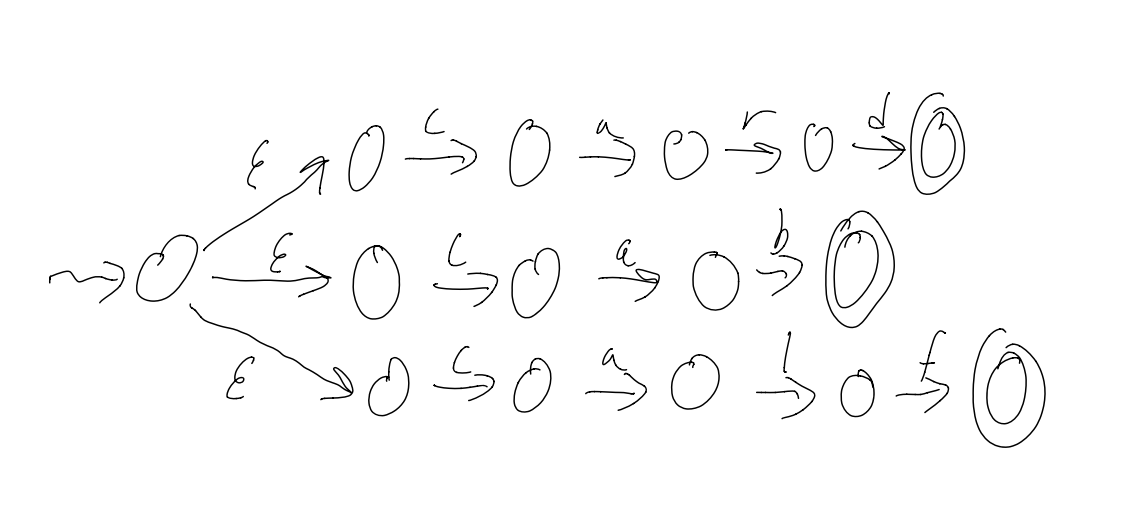## Converting ϵ$\epsilon$-NFAs to NFAs

Converting a ϵ$\epsilon$-NFA to a NFA is always possible.
There are a few ways to do this:
1) take shortcuts: ϵX=X for XΣ$\epsilon X = X\ for\ X \in \Sigma$
2) Pull back accepting states
3) Remove ϵ$\epsilon$-transitions
4) Remove dead states (states that we can never get to)

# Regular Expressions

## Definition

Defined recursively: a Regular Expression (RE) is:

• $\emptyset$, or
• ϵ$\epsilon$, or
• a$a$ where aΣ$a \in \Sigma$, or
• E1E2 where both are REs, or (concatenation)
• E1|E2 where both are REs, or (Alternation / Union)
• E* where E is a RE (Repetition)

And there is an Order of Precendence for the last 3:
1) Repetition
2) Concatenation
3) Alternation

## RE examples

L = {cab,car,card}, Σ$\Sigma$= {a,b,c,…z}
We can make a Regex for this langauge in different ways:
— cab|car|card or
— ca(b|r(d|ϵ$\epsilon$))

L = {w | w contains an even number of a’s}, Σ$\Sigma$= {a}
— (aa)*

L = {w | w contains an even number of a’s}, Σ$\Sigma$= {a,b}
— b*(ab*ab*)*

## Unix Shorthands and Extensions

Most Regex “engines” accept the following shorthands as valid syntax:
- [a-z] translates to a|b|c|…|z (the english alphabet)
- E+ translates to EE* (1 or more)
- E? translates to (E|epsilon) (zero or one)
There are plenty more!

# Lecture 11

## RE to ϵ$\epsilon$-NFA

We convert REs to ϵ$\epsilon$-NFAs piece by piece.

To concatenate E1 and E2 (making E1E2):
1) Add epsilon transitions b/w the end states of E1 and the start state of E2
2) Make the end states of E1 into regular states (we don’t want to accepts just E1 valid things anymore)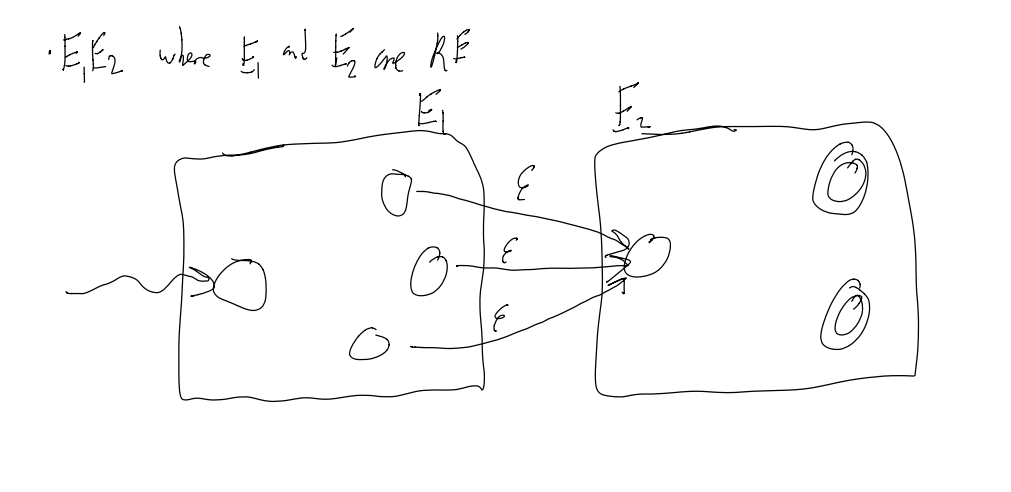To make an alternation between E1 and E2 (making E1|E2):
1) Add a external start state that has an epsilon transition to both start-states of E1 and E2To make a repetition from E1 (making E1*):
1) Add epsilon transitions b/w the end states back to the start state.
2) Make the start state an acceptance condition (to allow for no input)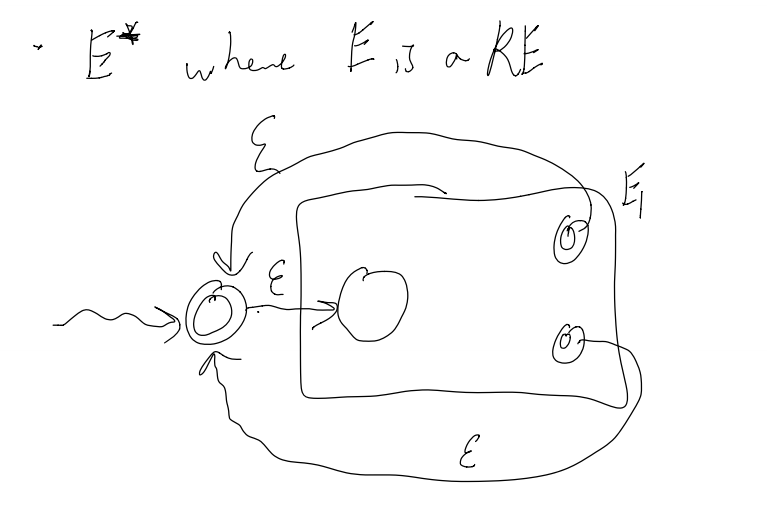We can take these ϵ$\epsilon$-NFAs and convert them to regular old NFAs using the same methods as before.

## Regular Languages

A Regular Language is a language which is either:
- specified by a Regular Expression
- recognized by an ϵ$\epsilon$-NFA
- recognized by an NFA
- recognized by a DFA
Note: all of the above can be converted to any other one

## Practical Application of DFAs

Most real-world examples do not care about recognizers (DNA match may be an exception)

Mostly, DFAs are used for:
- transforming / transducing input
- searching in text (the most common use of Regex)
- scanning / translating

## Transducers

A transducer is a DFA with output, that is, transitionslook like input/output vs just input

Example 1:
Removing stutters from Σ$\Sigma$ = {a,b}
So, aaabbaa -> aba, or baabbbb -> bab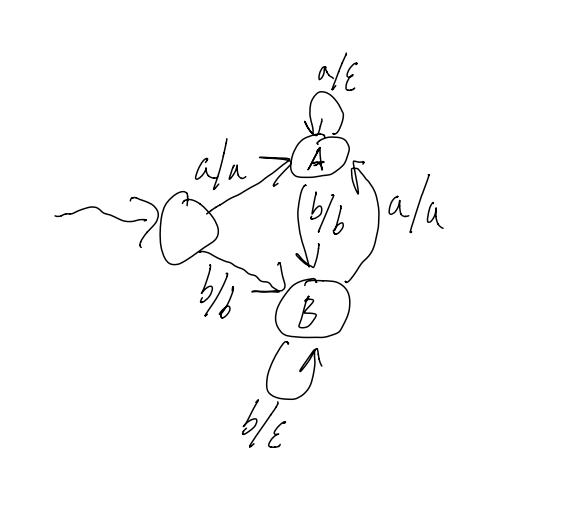Recall that there can be 2 types of Transducers: Moore Machines and Mealy Machines.
Moore machines output on leaving a state, Mealy machines output on transition

Notice how this is very similar to ECE-124…

## The Scanning Problem

Sometimes, it is easy to translate an input into an output:
Input: add $1,$2, $3 Output: <ADD><REGISTER><COMMA><REGISTER><COMMA><REGISTER> But what if we get something like this? Input: 0x1234abcd The output can be (theoretically be) both: Out1: <HEXINT> Out2: <INT><ID><INT><ID> How to we ensure we only get option 1? Well, we use the following algorithm. ## Simplified Maximal Munch Input is a word c1c2…ck i is the index of the current character i = 0 state = START loop newstate = ERROR if i < k: newstate = transition(state, c[i]) if newstate == ERROR: if state is not a final state: report error and exit if state is not WHITESPACE: output appropriate token state = START if i <= k: exit else: state = newstate i = i + 1  TL;DR: consume as much of the string in the current state as possible. ### MIDTERM MATERIAL ENDS HERE # Context Free Languages ## Big picture of Compilation There are 4 steps to compiling code: 1) Lexical Analysis 2) Syntactic Analysis 3) Context-sensitive (semantic) analysis 4) Synthesis (code generation) Other points: - Staging can improve error messages, (staging means using the “right tool for the job”) - Doing extra work at an early stage is possible, but may be over complicating. - Basically, have seperate programs in a pipeline: Input -> SCAN makes tokens -> PARSE makes parse tree -> CODEGEN makes MIPS code ## Non-Regular Languages What if we wanted to make a DFA over Σ$\Sigma$ = {a,b} for L = {w | number of a’s in w == number of b’s in w} It’s impossible! It’s infinite! No Deterministic Finite Automota can be Infinite! ## Context-Free Langauges In this case, L is not a regular language, it is context-free! These langauges are important to compilers for doing things like matching brackets, where we need to know that there is the same ammount of 2 different chars. Context-Free Languages are built from: • Finite sets • Concatenation • Union • Recursion (strictly more powerful than repetition) Recognizers for regular langauges use a strictly finite ammount of memory All it has to do is Remember the current state, and have a list of all the states with transitions. Recognizers for context-free langauages use a finite ammount of memory AND one (unbounded) stack (DFA + Stack <=> Parser) ## Context-Free Grammars Grammars are a way to specify Context-Free langauges. So, for example, here is a CFG: (S is start state) 1. S -> aSb 2. S -> D 3. D -> cD 4. D -> epsilon  These are called production rules, and together they make a specific grammar G. One such word that arises from these rules is accb S =1=> aSb =2=> aDb =3=> acDb =3=> accDb =4=> accb ## Terminology G = A context-free grammar (CFG) L(G) = Set of words specified by G (i.e: the langauge specified by G) a word = Sequence of tokesn that can be derived by G a derivation = Sequence of rewriting steps from G (informally) alternation = S -> aSb or S -> D concatenation = aSb recursion vs repetition = S -> aSb ## Formal Definition A context-free grammar (CFG) consists of: • N - A finite set of non-terminals (“not ending”) • T - A finite set of terminals (“ending”) • P - A finite set of production rules (rewriting rules) of the form Aβ$A \rightarrow \beta$ where AN$A \in N$, β(NT)$\beta \in (N \cup T)$* • S - A start symbol, SN$S \in N$ (by definition, it is always at the lefthand side of the first rule) So, from the example: • N = {S, D} • T = {a, b, c} (not ϵ$\epsilon$ though) • P = {the 4 rules} • S = S (can be called anything though) # Lecture 12 ## Example - Balanced Parentheses Example Words: ϵ$\epsilon$, (), (()), ()(), … CFG: 1. B -> (B) 2. B -> BB 3. B -> epsilon  Sample Derivation of (()()): B =1=> (B) =2=> (BB) =1=> ((B)B) =3=> (()B) =1=> (()(B)) =3=> (()()) ## Example - Binary Expressions G1 Words are composed of binary numbers (no leading zeros, other than 0) with + or - signs in infix notation Example words: 1001, 10+1, 11-11110+0, … CFG: let E = expression let B = binary number let D = non-zero binary number 1. E -> E + E 2. E -> E - E 3. E -> B 4. B -> 0 5. B -> D 6. D -> 1 7. D -> D0 8. D -> D1  Sample Derivation of 10 + 1 E =1=> E + E =3=> B + E =5=> D + E =7=> D0 + E =6=> 10 + E =3=> 10 + B =5=> 10 + D =6=> 10 + 1 This is called a Leftmost Derivation, since we always operate on the leftmost symbol. Rightmost is the opposite. ## Formal Definitions We say that αAβ$\alpha A \beta$ directly derives αγβ$\ \alpha \gamma \beta$ if there exists a production rule Ay$A \rightarrow y$. This is also called a Derivation Step We say that αAβ$\alpha A \beta$ derives αγβ$\ \alpha \gamma \beta$ if there are some possible derivation steps that can be preformed to get one from the other. Formally: αAβ=>$\alpha A \beta =>$* αγβ$\alpha \gamma \beta$ When there are two or more non-terminals which can be rewritten in a derivation, we pick the left-most/right-most non-terminal for rewriting Note: there are derivations which are neither left-most or right-most • G derives wT$w \in T$* if S=>$S =>$*w$w$ (eg: 10+1 is derived by G1) • L(G) = {w | S=>$S =>$*w$w$ and wT$w \in T$* } • L is context-free if there exists a CFG G such that L(G) = L ## Derivations as Proofs In a regular language, to prove a DFA M accepts a word W, we could give the sequence of states visited (but this is not often done as a proof) In a context-free langauge, to prove w is in a CFG G, we list our derivation steps as our “proof” ## Parse Trees Example: 10+1  E / | \ E + E | | B B | | D D | \ | D 0 1 | 1  Leaves are the terminals of our CFG The root is the start S This tree encapsulates all possible derivations of 10+1 in this grammar ## Meaning of the Parse Tree “Recursive Descent Parsing” / “Syntax Directed Translation” is when you compute the value of children to compute the value of their parent 10+1 means 3 (= 2 1) D -> 1 means D.val = 1 D_1 -> D_20 means D_1.val = D_2.val * 2 E_1 -> E_2 + E_3 means E_1.val = E_2.val + E_3.val  ## Problem: Grammars can encounter Ambiguity A real-world example of ambiguity would be “Sally was given a book by Joyce”. Does this mean that Joyce gave Sally a book, or Joyce wrote the book Sally was given? Formally: A String x is ambiguous if x$x \in$ L(G) AND there is more than one parse tree for x A CFG G is ambiguous if some word x$x \in$ L(G) is ambiguous Consider the binary expression grammar, and the string 1-10+11 There are two possible trees depending on if you apply rule 1 or rule 2 first! Some terminology for Ambiguity: A Grammar is ambiguous if there is a word x such that x has: -$\geq$ 2 different parse trees -$\geq$ 2 different left-most derivations -$\geq$ 2 different right-most derivations ## Fixing ambiguity in G2 Let’s rewrite our binary expression grammar differently: Instead of rules 1 and 2 being 1. E -> E + E 2. E -> E - E  We can rewrite them as: 1. E -> B + E 2. E -> B - E  When there is both Left and Right recursion in one rule, ambiguity is introduced. ## Associativity The string 1-10+11 means 1-2+3 == (1-2)+3 = 2 In our current grammar, if we try to use Recursive Descent Parsing to calculate the value of our expression from our parse tree, we actually end up with the incorrect answer -4, since our parse tree would result in the incorrect associativity of 1-(2+3) ### Fix To fix this, we need to once again change our first 2 rules… The rules 1. E -> B + E 2. E -> B - E  result in right associativity since they are right-recursive To fix these rules, we have to make rules 2 left associative 1. E -> E + B 2. E -> E - B  ## Precedence Problems What if we wanted to add multiplication to our grammar? Maybe it’s as easy as adding the rule E -> E * B? Well, consider 1*10+11… that works out nicely. Things fail when we then try 1+10*11, since we will eval the + before the *! ### Fix Nearer to the root -> later evaluation -> lower precedence Let’s add some rules: let S = Sum (new start state) let P = Product 1. S -> S + P 2. S -> S - P 3. S -> P 4. P -> P * B 5. P -> P / B 6. P -> B 7. B -> 0 8. ...  With these rules, our first expansion nearer to the top of the tree would be addition, and only later on would we expand products (thereby evaling them first) ## Regular Languages are Context-Free Langauges Why? Because. I didn’t copy the example because my laptop battery died. Just trust me, they are. # Lecture 13 # Top Down Parsing ## Parsing Given a grammar G and a word w,how can we find a derivation for w? There are 2 strategies: 1) Top Down: Find a non-terminal and replace it with the right-hand side of a rule (what we have been doing, eg: S => S + P => B + P) 2) Bottom Up: Replace a right-hand side with a non-terminal (eg: B + B * B => B + P) In both strategies, we have to make the correct decision at each step. ## Parsing Algorithm - Backtracking • Try each rule in turn • If we can move “forward,” do so • if we cannot move “forward,” go back a step and try the next rule • Stop when we find the derivation This works, but is not practical. It is exponential time wrt the number of rules… There are 2 different, better O(n) algos ## Parsing Algorithm - Stack Based Parsing For top-down parsing, we use a stack to remember information about our derivations and/or processed input Recall that a Context-free language can be recognized with a finite control (DFA) and one stack EG: balanced parens: - ( = push - ) = pop If the stack is non-empty at the end, then there is a faliure. ## Augmenting Grammars Empty words and stacks can cause hassles We augment our Grammars by adding “beginning” and “ending” characters 1. S' -> ├S┤ 2. S -> AyB 3. A -> ab 4. A -> cd 5. B -> z 6. B -> wz  ## Top-Down parsing with a Stack Invariant: Derivation = input already read + stack (read from top to bottom) Example of ├abywz┤ Derivation input read input to read stack actions S' ϵ$\epsilon$ ├abywz┤ S' Rule 1 ├S┤ ϵ$\epsilon$ ├abywz┤ ├S┤ match ├ ├S┤ ├ abywz┤ S┤ Rule 2 ├AyB┤ ├ abywz┤ AyB┤ Rule 3 ├abyB┤ ├ abywz┤ abyB┤ match a ├abyB┤ ├a bywz┤ byB┤ match b ├abyB┤ ├ab ywz┤ yB┤ match y ├abyB┤ ├aby wz┤ B┤ Rule 6 ├abywz┤ ├aby wz┤ wz┤ match w ├abywz┤ ├abyw z┤ z┤ match z ├abywz┤ ├abywz ┤ ┤ match ┤ ├abywz┤ ├abywz┤ ϵ$\epsilon$ ϵ$\epsilon$ ACCEPT! Observations: How do we apply these rules? What does expand mean? Use a rule: pop stack (LHS) or Push RHS (in reverse) How do we know when we are done? Accept when the stack is empty and the input is empty, reject otherwise how do we know which rules to use? Crystal ball? ## LL(1) Parsing We need: Predict(A,x) = A -> a so long that: 1) A is on the top of the stack 2) x is the first symbol of input to be read Where A is non-terminal (in N), and x is terminal (in T). Definition of an LL(1) Grammar: For all AN, xT, |Predict(A,x)|1$A \in N,\ x \in T,\ |Predict(A,x)| \leq 1$ Meaning of LL(1): L - left-to-right input L - leftmost derivation (1) - one token of “look ahead” (in terms of input) ## Constructing a Predictor Rable from Search Trees Recall the CFG: 1. S' -> ├S┤ 2. S -> AyB 3. A -> ab 4. A -> cd 5. B -> z 6. B -> wz  Let “.” be an error COLS are input (x). ROWS are non-terminating chars (A) a b c d y w z ├ ┤ S’ . . . . . . . 1 . S 2 . 2 . . . . . . A 3 . 4 . . . . . . B . . . . . 6 5 . . Adding a new rule is easy! Let’s add B -> epsilon We just change ROW:B - COL:┤ from an error to using rule 7 ## Algorithm for constructing a Predictor Table Below: α,β(NT)$\alpha, \beta \in (N \cup T)$* ; x,yT;AN$x,y \in T; A \in N$ Empty(α$\alpha$) = true if α$\alpha$ =>* ϵ$\epsilon$ can α$\alpha$ disappear? First(α$\alpha$) = {x | α$\alpha$ =>* xβ$\beta$} starting from α$\alpha$, what can I generate as the first terminal? Follow(α$\alpha$) = {y | S’ =>* α$\alpha$Ayβ$\beta$} starting from the start symbol, does the terminal y ever appear following a non-terminal A? Predict(A,x) = { A -> α$\alpha$ | x $\in$ First(α$\alpha$) } $\cup$ { A -> β$\beta$ | x $\in$ Follow(A) and Empty(β$\beta$) } ## LL(1) Parsing Algorithm Input: w push S' for each x in w: while (top of stack is some A in N): pop A if Predict(A,x) = {A -> a}: push a else: reject pop c if c != x: reject accept w  ## Non LL(1) Grammars: Consider the grammar: 1. S -> ab 2. S -> acb  We cannot tell if we should use rule 1,2 in the case where we have an S followed by a a. If we could look ahead 2 chars, we would be able to tell… We can actually conver this grammar into an LL(1) by changing the rules to 1. E -> aX 2. X -> b 3. X -> cb  ## Non LL(1) Language L = {anbm | n >= m >= 0} Grammar (ambiguous): 1. S -> epsilon 2. S -> aSb 3. S -> aS  Grammar (unambiguous): B is "balanced a and b" U is "unbalanced a and b" 1. S -> B 2. S -> U 3. B -> epsilon 4. B -> aBb 5. U -> aB 6. U -> aU  This language is actually not LL(k) for Any k! # Lecture 14 ## Bottom-up parsing (LR parsing) In LL/top-down parsing we have - input processed + stack = current derivation - note that the stack is read from top to bottom In LR/bottom-up parsing on the other hand, we hace -stack + input to be read = current derivation -stack is read from bottom to top ## A Trace of ├abywz┤ Derivaiton Stack Input Read Unread Input Action ├abywz┤ ϵ$\epsilon$ ϵ$\epsilon$ ├abywz┤ Shift ├ ├abywz┤ ├ ├ abywz┤ Shift a ├abywz┤ ├a ├a bywz┤ Shift b ├abywz┤ ├ab ├ab ywz┤ Reduce A -> ab ├Aywz┤ ├A ├ab ywz┤ Shift y ├Aywz┤ ├Ay ├aby wz┤ Shift w ├Aywz┤ ├Ayw ├abyw z┤ Shift z ├Aywz┤ ├Aywz ├abywz ┤ Reduce B -> wz ├AyB┤ ├AyB ├abywz ┤ Reduce S -> AyB ├S┤ ├S ├abywz ┤ Shift ┤ ├S┤ ├S┤ ├abywz┤ ϵ$\epsilon$ Reduce S' -> S S' S' ├abywz┤ ϵ$\epsilon$ ACCEPT Shift: moving a token from one place to another (push) Reduce: size of the stack may be rediced (pop RHS, push LHS) ## Shift/Reduce Somehow we shifted at just the right time, and reduced just at the right time. How did we know this? Recall that for LL(1) parsing, we had a predictor table For LR(1) parsing, we have an oracle, in the form of a DFA ## Constructing a DFA Oracle for LR(1) Grammars This is difficult to do. Donald Knuth proved a theorem that for any LR(1) grammar, you can construct a DFA (really a transducer) that tells us when to shift or reduce. But this doesn’t mean it’s easy…. ## Building an LR(0) Automaton Definition: An item is a production rule with a dot ($\bullet$) somewhere on the RHS (which indicated a partially completed rule) How to construct it: 1) Make the start state the first rule, with the dot ($\bullet$) in front of the left-most symbol of the RHS 2) For each state, label an arc with the symbol that follows $\bullet$ and advanced the $\bullet$ one position to the right in the next state 3) If the $\bullet$ preceded a non-terminal (e.g: A), add all productions with that non-terminal A on the LHS to the current state, with the $\bullet$ in the leftmost position ## Example: Consider this Grammar: S' -> ├E┤ E -> E + T E -> T T -> id  It catches sequences of additions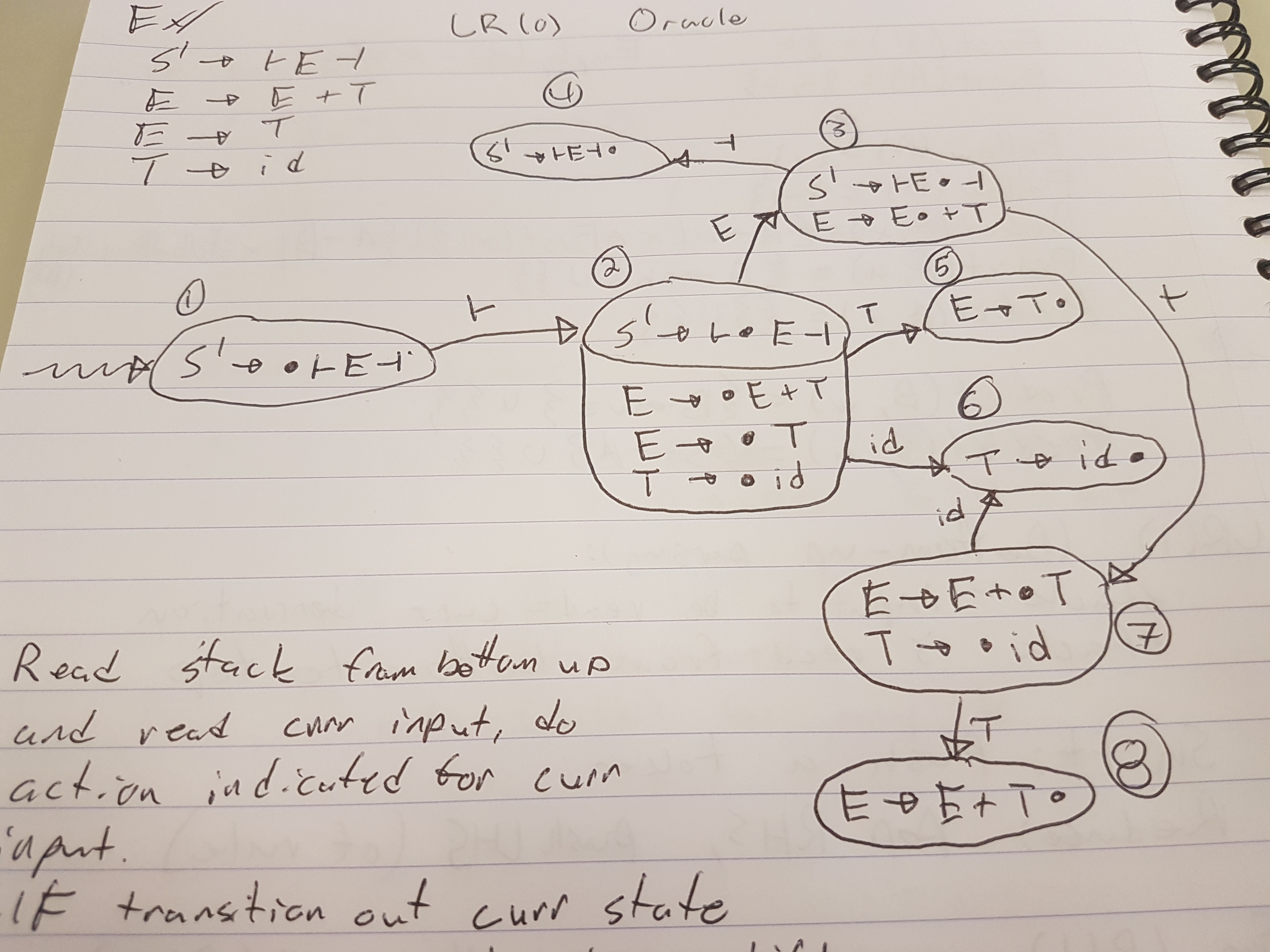#### Image brought to you by: James Hageman ## Using the Automaton For each input token: Start in the start state Read the stack (from the bottom up) and read the current input and do the action indicated for the current input If there is a transition out of our current state Shift (push) that input onto the stack We know that we can reduce if the current state has only one term and (dot) is the rightmost symbol Reduce (pop) the RHS off tehe stack reread the stack (from bottom up) follow teh transitions for the LHS push the LHS onto the stack Accept if S' is on the stack when all input is read  ## Using the Transducer Example ├id+id+id┤ Stack States Visited Input Read Unread Input Action ϵ$\epsilon$ 1 ϵ$\epsilon$ ├id+id+id┤ Shift ├ ├ 1, 2 ├ id+id+id┤ Shift id ├id 1, 2, 6 ├id +id+id┤ Reduce T -> id ├T 1, 2, 5 ├id +id+id+id┤ Reduce E -> T ├E 1, 2, 3 ├id +id+id+id┤ Shift + ├E+ 1, 2, 3, 7 ├id+ id+id┤ Shift id ├E+id 1, 2, 3, 7, 6 ├id+id +id┤ Reduce T -> id ├E+T 1, 2, 3, 7, 8 ├id+id +id┤ Reduce E -> E+T ├E 1, 2, 3 ├id+id +id┤ Shift + ├E+ 1, 2, 3, 7 ├id+id+ id┤ Shift id ├E+id 1, 2, 3, 7, 6 ├id+id+id ┤ Reduce T -> id ├E+T 1, 2, 3, 7, 8 ├id+id+id ┤ Reduce E -> E + T ├E 1, 2, 3 ├id+id+id ┤ Shift ┤ ├E┤ 1, 2, 3, 4 ├id+id+id┤ ϵ$\epsilon$ Reduce S' -> ├E┤ S' 1 ├id+id+id┤ ϵ$\epsilon$ ACCEPT ## What can go wrong? A Problem… What if the state looks like this: A -> a(dot)cb B -> g(dot)  Do we try to shift the next char, or do we reduce This is called a shift reduce conflict Alternatively: What if the state looks like this: A -> a(dot) B -> b(dot)  do we reduce by A -> a, or do we reduce by B -> b This is known as a reduce reduce conflict If any item A -> a(dot) occurs in a state in which it is not alone, then there is a shift-reduce or reduce-reduce conflict, and that means that the grammar is not LR(0) # Lecture 15 ## Example with Conflicts Consider right-associative expressions. Modifying our grammar slightly to allow this (i.e: reverse RHS of second rule) S' -> ├E┤ E -> T + E E -> T T -> id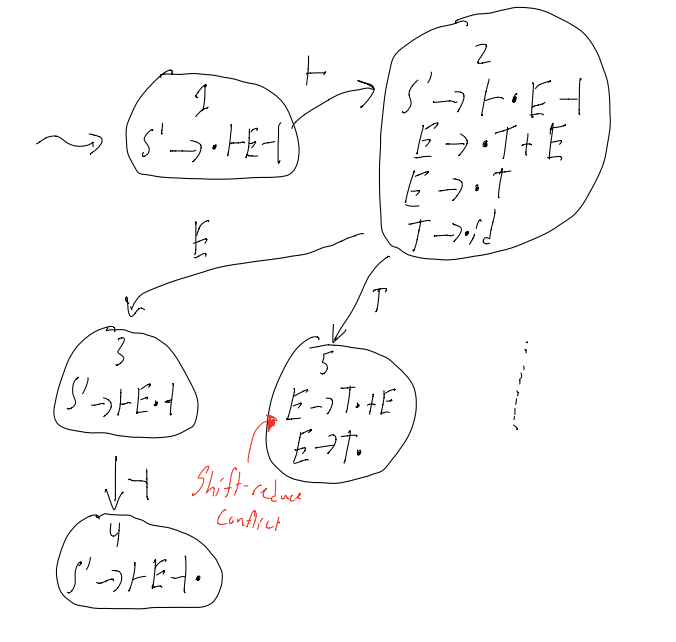## Parsing with Conflicts Suppose we are parsing a string that looks like ├id... Question: Should we reduce E -> T? Answer: it deptends… if input is ├id┤, then yes if input is ├id+..., then no ## SLR(1) Parser When we add this one lookahead character of a lookahead, we have an SLR(1) parser (SLR(1) = Simple LR with 1 character of lookahead) SLR(1) resolves many, but not all, conflicts - LR(1) parsing is more sopfisticated than SLR(1) parsers - LR(1) parses strictly more grammars - LR(1) automation is more complex - LR(1) and SLR(1) are identical parsing algorithms, the only difference is in the respecting outomation they create There is also a parser called LALR(1) which falls between SLR(1) and LR(1). LALR(1) = Lookahead LR(1) ## Making this more efficient Current running time of this algorithm: O(|w|2) Instead of scanning the stack each time, we can instead store (input, state) on the stack Start the transducer in state @ the top of the stack Running time is not only O(|w|) ## Outputting a Derivation In top down parsing: output the rules used to expand by In bottom-up parsing: It’s simple: every thime we do a reduction, just output the rule. BUT this isn’t quite right, since Derivations should start with the Start symbol, but with Bottom Up parsing, this is not the case… A Simple Observation Didn’t we say that this was LR(1) parsing? Yep! LR(0) and SLR(1) are both contained within LR(1) Doesn’t “R” mean rightmost derivation? Yep! Aren’t ew always reducing the leftmost non-terminal? Yes, but that is hard to see. Notice the direction we are creating the derivation… Write the derivation in reverse. ## Outputting the Parse Tree Create a “tree stack” Each time we reduce, pop the right hand side nodes from the tree stack push the left-hand side node and make its children the nodes we just popped Example: ├id+id+id┤## Going Back Looking at L = {anbm | n >= m >= 0} (the non LL(k) langauge) 1. S' -> ├S┤ 2. S -> aS 3. S -> T 4. T -> aTb 5. T -> \epsilon  What do we do when we see the symbol: ├ - shift a - shift b - reduce by 5 (only on the first b), then shift, then reduce by 4 ┤ - reduce by 3, repeatedly reduce by 2, shift, reduce by 1 EG: ├aaaabb┤## Fun Facts Theorum: For any augmented LR(1) grammar, there is an equivalent LR(0) grammar Theorem: The class of languages that can be parsed deterministically with a stack can be represented with an LR(1) grammar # Lecture 16 ## Big Picture of WLP4 Compilation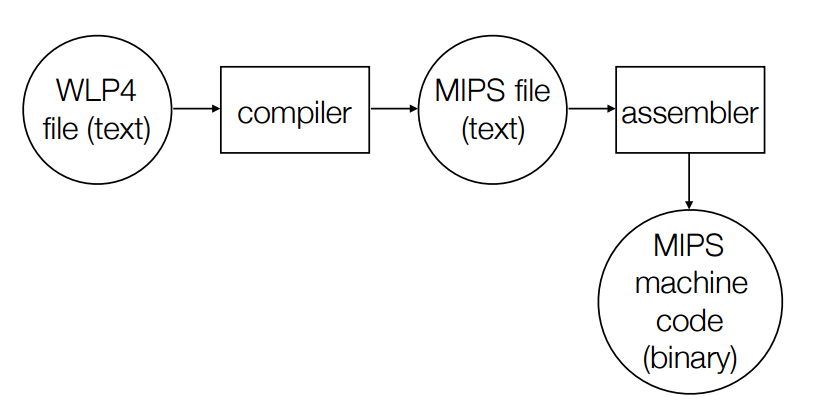#### Zooming into the compiler: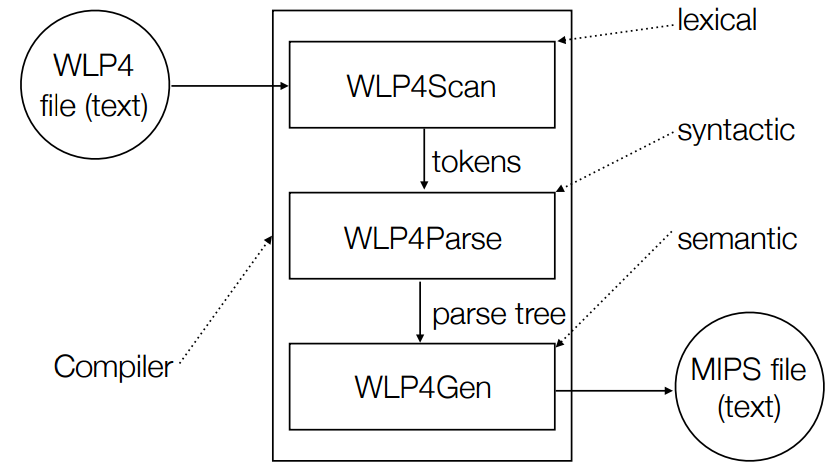Recall: Lexical = If you are using valid words (not neccessarily order) Syntactic = If you are using the right words in the right order Semantic = If you are using the right words in the right order with respect to other special rules ## Example: Consider the input file int wain (int a, int b) { return a; }  The tokens we get from the scanner would be: INT WAIN LPAREN INT ID COMMA INT ID RPAREN LBRACK RETURN ID SEMI RBRACK  The parse tree might would thus look like: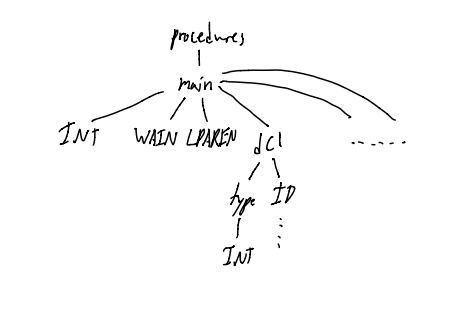## Context Sensitive Analysis Where the statement occurs matters Input: parse tree (therefore, the program is already syntactically valid) Output: If the parse tree is semantically incorrect, then error, otherwise, output the same parse tree… Basically: This is just a “check” step You can optionally “add” some info to the outputted parse tree that makes code generation easier ## Possible Errors: If a program is syntactically valid, what else can go wrong? Variables: Types Variables: Dupliacte Variables: Undeclared Procedures: Undeclared Procedures: Duplicate Procedures: Types of paramerters and return values Procedures and Variables: type of calling vars must match procedure decl. type Variables and Procedures: scope of variables in/out of procedures ## Solving the “Variable Declaration” Problems We’ve done this already! Just use a Symbol Table! This solves undeclared and duplicate variable problems… We can also solve the variable type problem with the symbol table by adding a column to the table for the type of the variable… So, 3 cols: | name | location | type | Consider: int wain(int a, int b) { return x; }  x is undeclared, and we can catch this by checking if x is int the symbol table when it is used Consider: int wain(int a, int a) { return a; }  a is duplicated, and we can catch this by checking for if a var is already in the symbol table when it is attempting to be defined. ## Procedures Bite Us consider: int wain() { int x = 0; return 1; } int wain(int a, int b) { int x = 0; int y = 0; y = g(); return 1; }  There are several things wrong here: 1) “false positive” on x being a duplicate variable (when using a global symbol table) 2) undefined function g() 3) duplicate procedure names (wain x2) ## Solving “Duplicate / Undeclared” Procedures & Variables At the Same Time For each procedure, we make it’s own symbol table Make a global symbol table for procedures - table should hold | name | type | param types | ## Obtaining Signatures Signature: parameter types, and # of parameters To get this info, we can leverage our grammar: paramlist -> dcl paramlist -> dcl COMMA paramlist dcl -> type ID params -> \epsilon  dcl will eventually give us the type! if params is empty tho, then that means we have an empty signature Note: in WLP4, the only return type is int, so that makes life a bit easier ## Why Types Matter Recall: looking at bits doesn’t actually tell us what they represent… Types help us remember what exactly a variable means So, if we had code like int* a = NULL; a = 7 // should probably be prevented...  In WLP4, there are only 2 types: int and int* ## Solving the “Type Mismatch” Problems See the “WLP4 semantic rules” handout from the website Notation: assumptionsconsequences$\frac{assumptions}{consequences}$ To type check: - make sure that all the rules in the handout are met wehn computing the type of an expression - Make sure that the left hand side type (lvalue) is the same as the right hand side type (expr) Hint: pointers make life more “interesting” And as always: if it’s not in the rules sheet, it ain’t valid! ## Types of Indentifiers Look in my symbol table for the type of a praticular ID Big goal of A8: Be able to typecheck an expression like x = t + r - s ## Addition and Subtraction are Hard int + int -> int int* + int -> int* int + int* -> int* int* + int* -> ERROR int - int -> int int* - int -> int* int - int* -> ERROR int* - int* -> int // offset between two addresses: # of elements between them  ## Follow the spec to the tee! There is a Spec Sheet of the 241 website that outlines EXACTLY how types can be used in all the various contexts! Follow it! NOTE: I switched to Troy Vasiga’s lecture section, so there might be shift in notes from this point… # Lecture 17 ## More advanced context-sensitive analysis “Real” languages add more complexity (see CS444/CS442) They have things like: • Scope • if-statements, while loops may have variable declarations • *in WLP4, all declarations are at the top of functions • User-defined types • classes, structs, objects • Nested Pointers • terrible things like *****a • OOP stuff • inheritance, overloading… All of this stuff adds a stupid ammount of complexity. Don’t take CS444/CS442 unless your body is ready. ## Code Generation for WLP4MPMP WLP4MPMP = Waterloo Language Plus Pointers Plus Procedures Minus Pointers Minus Procedures AKA WL ## Code Generation (A9, A10) Input: 1) Parse Tree (that is guaranteed to be Semantically Valid) 2) Symbol Table for Procedures and Variables Output: MIPS assembly language (equivalent to wlp4 program in terms of output and return values) Outputs will vary from person to person, since there are different ways to do the same things with MIPS assembly. ## Code Generation Issues • Correctness • guaranteed to generate code with the correct meaning • this is the minimum requirement • Ease of writing compiler • this is kind of language specific… • WLP4 is “easy” • C++ is not easy • Efficiency of the compiler • the speed of compilation • Namely, have it to be O(n) • O(n2) is a instafail (for WLP4) • Efficiency of the compiled code • how fast the generated code runs • this boils down to Optimizations • don’t focus on this until you have a compiler the WORKS ## Syntax Directed Translation Fundamental idea: traverse (go all the way down, and climb up) the parse tree and gather information. ## First Rule main -> INT WAIN LPAREN dcl COMMA dcl COMMA... becomes code(main) = <stuff> "+" code(dcl1) "+" code(dcl2) "+" code(dcls) "+" code(statement) "+" code(expr) "+" <more stuff>  "+" means that don’t actually do string concatenation (that becomes O(n2) in runtime, which is bad) TL;DR: Ya code is gonna be recursive! ## Three more simple rules statements -> epsilon becomes code(statements) = ""  expr -> term code(expr) = code(term)  term -> factor code(term) = code(factor)  In general: if a -> b then code(a) = code(b) where a and b are non-terminals ## A9P1 Recall that input must be semantically valid what do the WLP4 programs look like? int wain(int a, int b) { return a; // OR return b; }  That’s all of ‘em. The parse tree will thus look something like this: -------------------------------------- main -------------------------------------- | | | | | | | | | | | | | | INT WAIN LPAREN dcl COMMA dcl RPAREN LBRACE dcls statements RETURN expr SEMI RBRACE / \ / \ | | | type ID type ID epsilon epsilon term | | | INT INT factor | ID  What do the equivalent MIPS programs look like? Assuming 1st param is in$1, and 2nd param is in $2, and return value is placed in$3

Well, it’s either

add $3,$1, $0 jr$31


or

add $3,$2, $0 jr$31


ggez

(theoretically, you can literally just write an if statement to solve this assignment question…)

## Changes to the symbol table

This table is generated in A8

Name Type Location
a int $1 b int$2

For all of A9: Type is always int, Locations are Registers…

## How to store variables

Option A: Variables in Registers
One variable per register, stored somehow in the symbol table.

Problems > 32 variables…

Option B: Variables in RAM using .word
Each variable x in WLP4 program corresponds to label x in MIPS

Example:
Ouptut MIPS code like

...
jr $31 ; allocated variables a: .word 0 b: .word 0 c: .word 0  Problems: Problem 1) Getting the value of c for example: lis$3        ; THESE LINES ARE OVERHEAD
.word c       ; (and lead to poop efficiency)
lw $3, 0($3)


This way of doing vars almost DOUBLES code length!

Problem 2) Name Clashing
In A10, there are multiple procedures that might have the same names…

## The best way to store variables

Option C: Variables on the Stack

Suppose we have n variables
How to get n? => Just look at the size of the symbol table!

Here is what we propose..
The Stack

|           |
|           |
-------------
| initial c |
-------------
| initial b |
------------- memloc in $29 | initial a | ------------- memloc in$30


The associated Symbol Table:

Name Type Location
a int 2
b int 1
c int 0

With this method, we can easily find the address of the ith variable, simply by doing $30 + 4 * i But, what about$30 having to change?

lets use another register, register $29$29 will be the frame pointer, and it will point to the “bottom” variable

Now, we can find the ith variable by doing $29 + 4 * i ## Some conventions Special Registers: •$0 = always 0, that we will treat as False
• $1 = value of 1st parameter, initially •$2 = value of 2nd parameter, initially
• $3 = return value •$30 = stack pointer
• $31 = return address Also, convention dictates that •$4 = 4
• $11 = 1 , that we will treat as true •$29 = Frame Pointer (where the variables are)

## Code Structure

Prologue:
Define all of the constants we need, and misc setup

lis   $4 .word 4 lis$11
.word 1
<store $31> <store variables / setup$29>


Generated Code:
self explanatory…

Epilogue
Cleanup

<restore $31> jr$31


## One More Rule (A9P2)

factor -> LPAREN expr RPAREN

now you can have code like

int wain(int x, int y) {
return (x); // or return ((x)), and so on...
}


This code will actually return the exact same MIPS as A9P1!

## A9P3 - Full Expressions

so, things like

int wain(int a, int b) {
return a + b;
}


are fair game.

That code might result in

add $3,$1, $2 jr$31


You can also have

int wain(int a, int b) {
return a + b + a;
}


How might the code for that look like?

Well, we have a rule expr -> expr PLUS term, so the parse tree looks something like this…

          _____ expr _____
/       |        \
__ expr __  PLUS      term
/    |     \            |
expr  PLUS  term        factor
|           |            |
term       factor       ID (a)
|           |
factor     ID (b)
|
ID (a)


Note that if we try and store the valie of the left-side of the tree in $3, and also do the same with the right hand side of the tree, the value in \$3 is overwritten!

Solution?

Use the stack!

expr1 -> expr2 PLUS term

code(expr1)  =   code(exp2) // $3 = value of expr2 + push($3)   // push $3 onto the stack // sub$30, $30,$4
//   sw  $3, 0($30)
+ code(term) // $3 = term + pop($5)    // pop from the stack into $5 // lw$3, 0($30) // add$30, $30,$4
+ "add $3,$5, $3"  # Lecture 18 # A9P4: Printing statements -> PRINTLN LPAREN expr RPAREN SEMI A First Attempt: code(statements) = code(expr) //$3 = value of expr
+ move $3 to$1
+ call print (a function we have)


Problems:
1) destroys $1 2) destroys$31

## Sidenote: Where is print?

We are given print in a merl file, so really, all we need is an import print in the Prologue

code(statements) =   code(expr)
+ "add $1,$3, $0" + "lis$5"
+ ".word print"
+ push ($31) + "jalr$5"
+ pop($31)  ## How do we not destroy$1

1) try different calling conventions?
eg: make print get it’s argument from $3 NO! Don’t do this! This is extremely bad! All previous code that uses print is broken! 2) save register$1 before and after calling print

Okay… but it adds a significant ammount of overhead. 4 instructions per call.

3) stop worrying: do nothing.

## A special note about parameters in $1 and$2

### They are not special.

We save these on the fram at 0($29) and -4($29) as soon as we see them, so there is no reason to care about $1 and$2 !

• Comment both the code you write, AND the code that you generate!
• When things go wrong, and the MIPS doesn’t work, having comments that help you understand what a chunk of MIPS is supposed to do will help a lot!
• Use a rule-based structure
• each grammar rule will represent one function/procedure/step in our compilation process
• the parse tree indicates which subtrees need to produce the code before the “entire” code has been produced
• test, test, test (or don’t, but in that case, good luck fam)

## Declarations (A9P5)

Adds the rule dcls -> dcls dcl BECOMES NUM SEMI
i.e: there can be as many declarations as we want.

Recall:

• Variables on the frame
• Symbol table stores all declared variables
• each variable has an offset (eg: ith var is located at $29 + 4*i) ## Giving variables values The code to do that BECOMES NUM SEMI part of the rule, we write some code like:  code(NUM) // basically, put the number in NUM into$3
+ "sw $3, 4*i($29)" // (calc 4*i when compiling using the SymbolTable)


Also, what about the rule statement -> lvalue BECOMES expr SEMI (for now, lvalue is just and ID, not pointers yet…)

code(statement) =   code(expr)
+ "sw $3, 4+i($29)" \\ i is the offset of the ID in the LHS


## Booleans (A9P6)

The only spot where booleans are allowed are in control structures (like if, while)

Rule: test -> expr1 LT expr2

Recall conventions:
$0 = 0 = false$11 = 1 = true

MIPS code:

code(test) =   code(expr1) // $3 = expr1 + push($3)
+ code(expr2) // $3 = expr2 + pop($5)
+ "slt $3,$5, $3"  ## While loop (A9P6) statement -> WHILE LPAREN test RPAREN LBRACE statements RBRACE MIPS code: code(statement) = "loopY:" + code(test) //$3 = test (0 or 1)
+ "bne $3,$11, endloopY" // if my test failed, end the loop
+ code(statements)
+ "beq $0,$0, loopY"
+ "endloopY:"

// for Y = 0, 1, 2 ... for every while loop in the program


There is a long range problem: what if we have a really long while loop…
Branches only let you jump +- 215 (approx 32 000) words

Solution: Instead of using beq $0,$0, label, do

lis $5 .word loop jr$5


## If statement (A9P8)

statement -> IF test ... statements1 ... ELSE ... statements2 ...

code(statement) =   code(test) // $3 is 0 or 1 + "bne$3, $11, elseX" + code(statements1) + "beq$0, $0, endIfX" + "elseX: " + code(statements2) + "endIfX: " // for X = 0, 1, 2 ... for every while loop in the program  ## Back to booleans (A9P7) test -> expr1 GT expr2 //we use this chunk of code a lot... A(a,b) = return code(a) + push($3)
+ code(b)
+ pop($5) code(test) = A(expr1, expr2) + slt$3, $3,$5


test -> expr1 NE expr2

code(test) =   A(expr1, expr2)
+ "slt $6,$5, $3" + "slt$7, $3,$5"
+ "add $3,$6, $7"  ## Finishing Booleans (A9P7) test -> expr1 EQ expr2 code(test) = code(NE) // (literally the code to do not equal) + "sub$3, $11,$3" // negates this


test -> expr1 GE expr2

code(test) =   code(LT)             // (literally the code to do less than)
+ "sub $3,$11, $3" // negates this  test -> expr LE expr code(test) = code(GT) // (literallt the code to do greater than) + "sub$3, $11,$3"


# Lecture 19

## Pointer Basics (A10P1)

factor -> NULL

Note: we want dereferencing NULL to Crash and Burn

code(factor) = "add $3,$11, 0"  If we dereference “1” we get a “unaligned word value” error, and that is good! factor1 -> STAR factor2 We know the type of factor2 is int*, and that factor1 is thus a int code(factor1) = code(factor2) //3 is some address
+ "lw $3, 0($3)"


factor -> AMP lvalue

Why, you might wonder, is the rule written this way, instead of factor -> AMP factor?

Well, we don’t want to be able to do things like:
- &5 or &NULL
-&&&x (since that would be of type int*** which is not allowed)

Considering what grammat lvalue can have:
1) lvalue -> ID - eg: x = ...
2) lvalue -> STAR factor - eg: *x = ...
3) lvalue -> LPAREN lvalue RPAREN - eg: (*x) = ...

Also, the grammar rules disallow:
- x + 2 = y - doing the algebric
- 7 = 4 - redefining constants

## Code for &

factor1 -> AMP lvalue

We actually need to do a look ahead at what lvalue is…

Three cases:
1) lvalue is an identifier (eg: &x)

code(factor1) =   "lis $3" + ".word <offset of ID>" + "add$3, $29,$3"


2) lvalue is a STAR factor2 - i.e: &*x
well, &*x == x… sooooo

code(factor1) = code(factor2)


3) lvalue is a LPAREN lvalue RPAREN

trivial


## Assignment to pointer dereference

lvalue -> STAR factor (eg: *x = 4 + 9)

Let’s step back and look at the rule:
statement -> lvalue BECOMES expr SEMI

if lvalue == ID do A9 stuff
if lvalue == STAR factor

code(statements) =   code(factor)     // $3 has an address + "add$6, $3,$0" // $6 has ^ addr + code(expr) //$3 has expr
+ "sw $3, 0($6)"


## A “simple” WLP4 Program

Running with mips.array (so $1 = base_adress,$2 = size)

int wain(int *a, int n) {
return *a;
}


Wat do?
Returns the value of the first element in the array.

How do?
Theoretically: “lw $3, 0($1)” (but not for realsies, since we throw $1 on the stack, blah blah blah) ## What mips.array is doing mips.array allocates memory on the heap and then calls wain with the address and the size as parameters ## A Second Example int wain(int *a, int n) { return *(a+1); }  Wat do? Returns the second element of the array. SIDENOTE: in higher level languages, we would do a, but that is EXACTLY EQUIVALENT to *(a + 1) (and by extension, also *(1 + a) and, if you’re a real sadist: 1[a]) ## Back to Compilation (A10P2) factor -> NEW INT LBRACK expr RBRACK — Create and Array statement -> DELETE LBRACK RBRACK expr SEMI — Reclaim an array How do? In Prologue: link in alloc.merl with a .import alloc.merl! This gives us access to a few functions: • init = initialize the heap • make sure to set$2 to
• 0 if wain takes (int, int)
• length of the array if wain takes (int, int*)
• new = allocates an array, and returns pointer to it in $3 • make sure to set$1 to the size of the array
• IF UNSUCCESSFUL: returns 0 (NOT NULL i.e 1)
• you sould make that into NULL tho
• delete = frees array
• make sure $1 is the base address of what you want to free • CHECK IF IT’S NULL, and don’t try to delete if it is! • you don’t neeeed to throw an error, just don’t do it # Lecture 20 ## Pointer Arithmetic expr1 -> expr2 PLUS term if expr2 is an int, and term is an int, do A9 stuff. if expr2 is an int*, and term is an int (or vice versa): code(expr1) = code(expr2) + push($3)
+ code(term)
+ pop($5) + "mult$3, $4" + "mflo$3"
+ "add $3,$5, $3"  You need to use register$5 in your mult and mflo if you have the reverse case

expr1 -> expr2 MINUS term

if expr2 is an int, and term is an int, do A9 stuff.

if expr2 is an int*, and term is an int (NOT vice versa):

code(expr1) =   code(expr2)
+ push($3) + code(term) + pop($5)
+ "mult $3,$4"
+ "mflo $3" + "sub$3, $5,$3"
// same as addition, but sub at the end...


if expr2 is an int*, and term is an int*:

code(expr1) =   code(expr2)
+ push($3) + code(term) + pop($5)
+ ... zoned out for a second...


## Comparison of Pointers (A10P4)

Aswe know, since semantic analysis passed, comparisons like test -> expr1 LT expr2 must have the SAME types from expr1 and expr2!

The only thing we actually need to change is that when we are doing pointer comparisons, we use sltu (unsigned) instead of slt (since you don’t want an out-of-integer boundary error)

## Procedures (A10P5+)

There is an important thing we have to do when we add procedures!
We must add a jr to the prologue to jump to wain, since the order of code generation is going to result in procedures being created first, and wain later, and as such, we have to jump to wain (we cannot assume that we will immediately begin executing in main anymore)

## What does each procedure need to do?

Each procedure has it’s own Prologue and Epilogue
Note: we still have out global pro/epilogue, these are seperate.

What should these contain?

## Prologue - Saving Registers

The overall (naiive) idea: just save + resore all the registers.
This would work… but it’s kind of (very) inefficient…

Better idea: just save + restore the important registers.
Namely: only save / restore $31 and$29

So, suppose procedure f calls procedure g. There are 2 approaches:

Caller Save: f (caller) saves all registers that contain important information before f calls g
Callee Save: g (callee) saves any registers which it modifies

What have we been doing so far?
Callee Save => We saved registers at the begining, and restore at the end
Caller Save => Saved $31, and restored it afterwards We actually have to so a bit of both! ## What about$29?

Suppose g (the callee) saves $29 i.e: g saves registers, and also sets$29 to g’s frame

This is tricky, since saving registers -> changes $30 -> set$29 to above $30 -> saved register below local variables -> things get complicated… Suppose f (the callee) saves$29

i.e: set $29 to the correct spot (the top of the stack) on entering g Verdict and MIPS outline: Use f of course! f: ... // f saves the things important to it push($29) // Where are my varaibles
push($31) // Where do I return to lis$5
.word g
jalr $5 pop($31)
pop($29)  ## Possible Errors… What if the name of a procedure matches that of a built in compiler procedure! eg: procedures like while0, or print, or new, etc… Well, simple fix: prefix all user-defined procedure names with a common prefix (essentially seperating the user-namespace and the compiler-namespace) So, for example, if a user defines a function print, we actually label it Fprint ## Parameters Let’s use Registers for Parameters! Nah! That’s a shit idea! What if we have more than 31 parameters… Alternatively, we could be smaht, and save our parameters on the Stack So, for factor -> ID LPAREN arglist RPAREN code(factor) = push($29)
+ push($31) + [ eval each arg + push arg onto stack ] + lis$5
+ .word <ID lexeme>
+ jalr $5 + "pop" the args (add to$30 so it's past the args)
+ pop($31) + pop($29)


## Code for Procedure

I zoned out again… 8:30 classes, amirite?

# Lecture 21

## Problems and Mathematical Fix

Parameters and local variables are Separated by Saved Registers
Soln: Save local variables before saving other registers

Old Symbol Table vs New Symbol Table:

consider function taking params a,b,c with local vars d,e,f
We need to fix the ST to work with the “params below $29” convention var old new a 0 3 b -1 2 c -2 1 d -3 0 e -4 -1 f -5 -2 How fix? EZ: just add the “number of parameters” to each value in the symbol table ## Alternate Fix? Could have also saved all the registers in the caller, but… then we would have to do a lot of saving and restoring for every procedure call. ## Optimization Not really tested => didn’t take notes. Fascinating stuff though :D OH CRAP. THIS IS BIENG TESTED. RIP. GLHF # Lecture 22 ## Runtime memory management There are 3 things to ocnsider when talking about memory management 1) initialization 2) allocation 3) reclamation On the stack, these 3 are done as follows: 1) initialization - MAGIC (don’t worry how$30 is set…)
2) allocation - push (decrease $30) 3) reclamation - pop (increase$30)

Heap usage is a bit harder…
1) initialization - init
2) allocation - new
3) reclamation - delete

How do these functions actually work?

The stack is easy, it only ever gets allocated once, and reclaimed at the end.
When allocating on the Heap though, there are many allocates and deletes, which leads to complications like Fragmentation

## Four dimensions of heap allocation

All of these are actually just Programming Language Design Decisions, i.e: the memory management model is not intrinsic to CS as a while, and can vary by langauge.

1) fixed vs. variable sized blocks
fixed = If you ask for memory, you get a fixed size of memory each time
variable = you can ask for many different sizes of memory

Variable has the downside of having no guaranteed perfect implementation

2) implicit vs. explicit allocation
3) implicit vs. explicit reclamation

implicit = allocation happens without programmers input (no new statements)
exmplicit = programmer explicitly satates how much memory they want, and then says when they are done with it.

Common combos of (im|ex)plicit allocation:
explicit / explicit: WLP4, C++, both have new and delete
implicit / implicit: Racket, Lisp
explicit / implicit: Garbage collected languages

implicit / explicit doesn’t really happen, since it would be wierd to have a program “magically” give you memory, and then force you to tell it when you are done with it…

4) language / implementation — Can pointers be relocated?

## An easy case

Fixed Size
Explicit Allocation
Explicit Reclamation
No pointer reallocation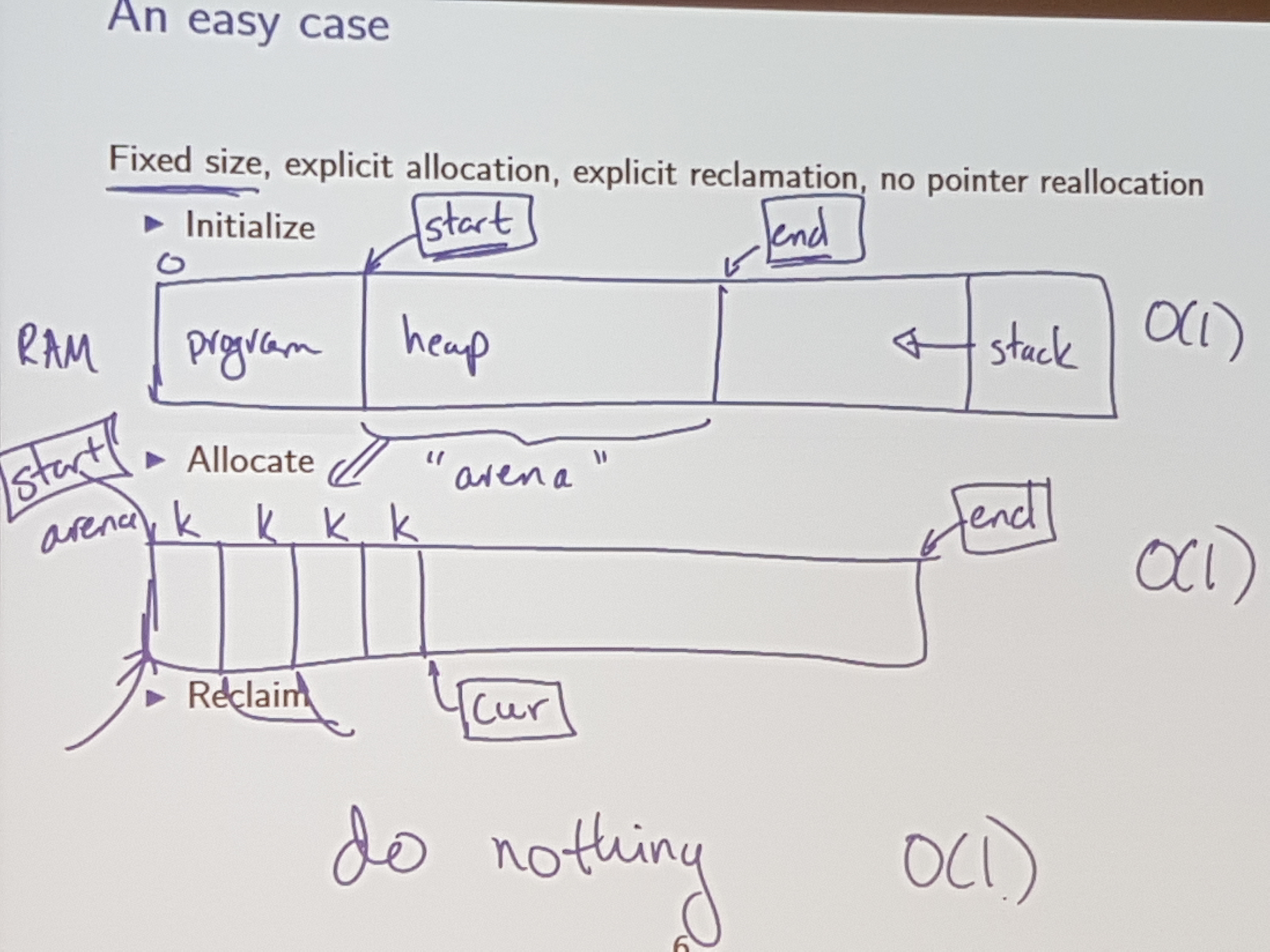Notice: Is a constant time op;

## Being slightly more clever

Instead of doing nothing, keep a free list (a list of blocks that have been freed)Back to allocate: with this change to reclamation

if freelist != empty: O(1)
- give up first block from free list

else if curr != end: O(1)
- give out curr and move curr ahead one

else: ERROR: OUT OF MEMORY

## Another way to implement this:

Use parts of each allocated block to keep track of the free list…Costs:

allocation: give block (3), set free to (2)
reclamation: set (4) to point to (3), set free to point to (4)
faliure: every block is allocated simultaneously
waste: no “real” waste, other than internal fragmentation

## Variable size blocks

Life only gets more difficult.

A Picture: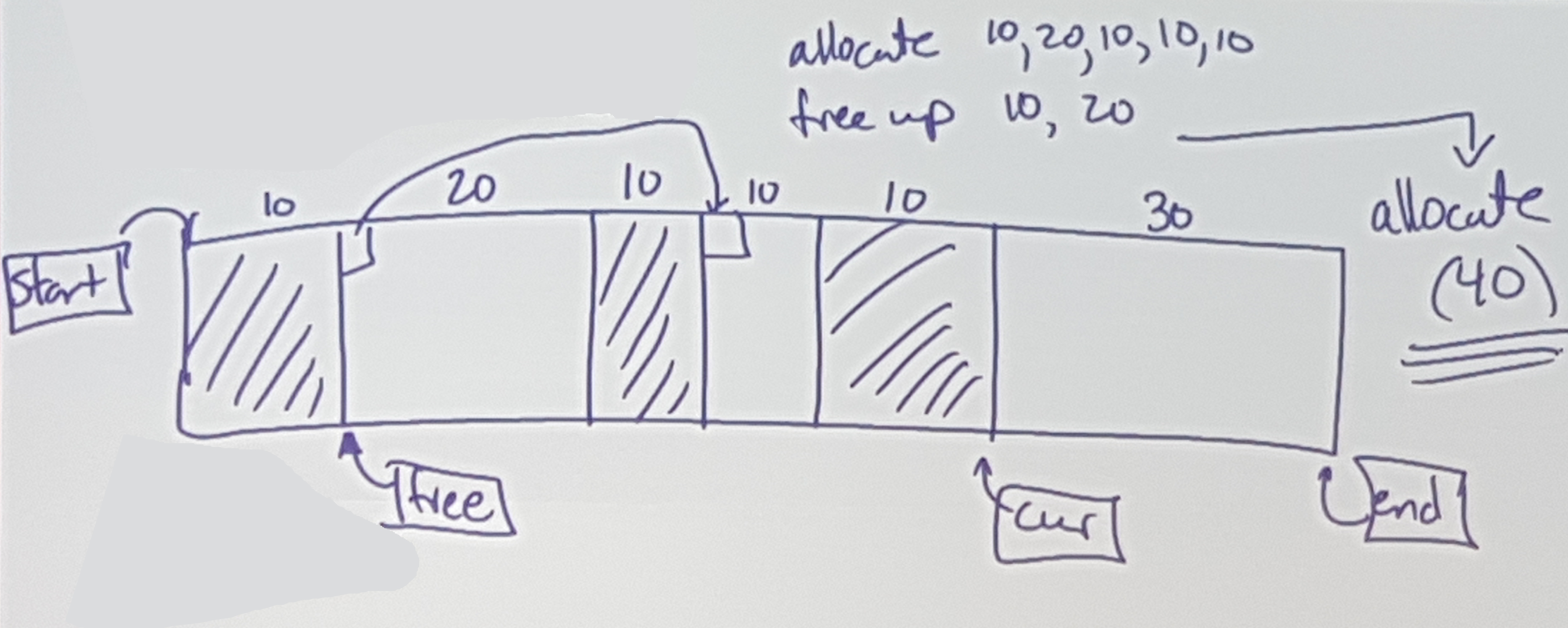Issues: Allocate 40 -> OUT OF MEMORY (even thought there are 60 free…)

Allocate:
We need to find a “hole” / “chunk” big enough

Reclaim:
Can we coalesce? Merge chunks together?

## Fragmentation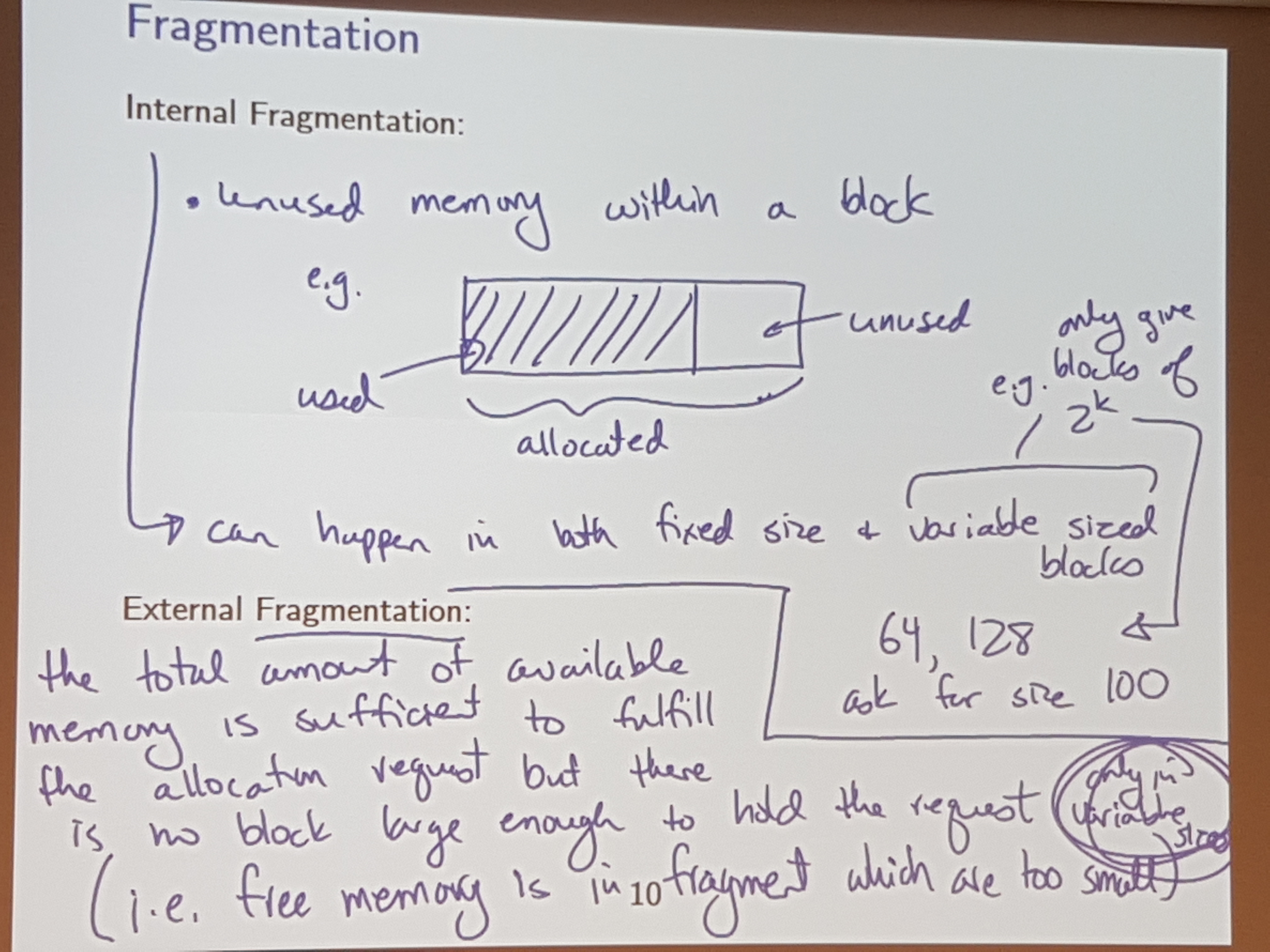## Allocation strategies: Description

First Fit: find the first available block which is big enough.
Best Fit: find the block which has the least ammount of space beyond the requirement
Worst Fit: find the block which has the most ammount of space beyong the requirement

## Allocation strategies: ExampleNo matter what strategy you use, none are perfect! There will always be sequences of allocates / deletes that fail on one strat, and work on antother!

## Allocation strategies: Implementation

First Fit: Search through the free list
Naiively: O(n)
Clever: O(log(n)) using a balanced tree / skip list

Best Fit: Find a “just big enough” piece
This is difficult (more complicated than first fit)

Also, the “waste” left behind in Best Fit is pretty useless, since it is usually “shavings” (really small blocks of memory), which usually doesn’t get allocated, and therefore, piles up.

Worst Fit: Find the biggest piece
Using a Max-heap (CS240 for dem deets)

## Deallocation

Extra information is required to help with merging.

So, when you ask for 10 words, alloc.merl actually uses 15, and stores some extra info before the “real” block:

0x00 Cookie “This is a start of a block”
0x04 Prev pointer to prev block
0x08 Next pointer to next block
0x0c Length size of block
0x10 Alloc flag (if currently allocated or not)
0x14 - ??? “real block” the actual useable alloc’d memory

# Lecture 23 - last lecture :(

## Four cases of delete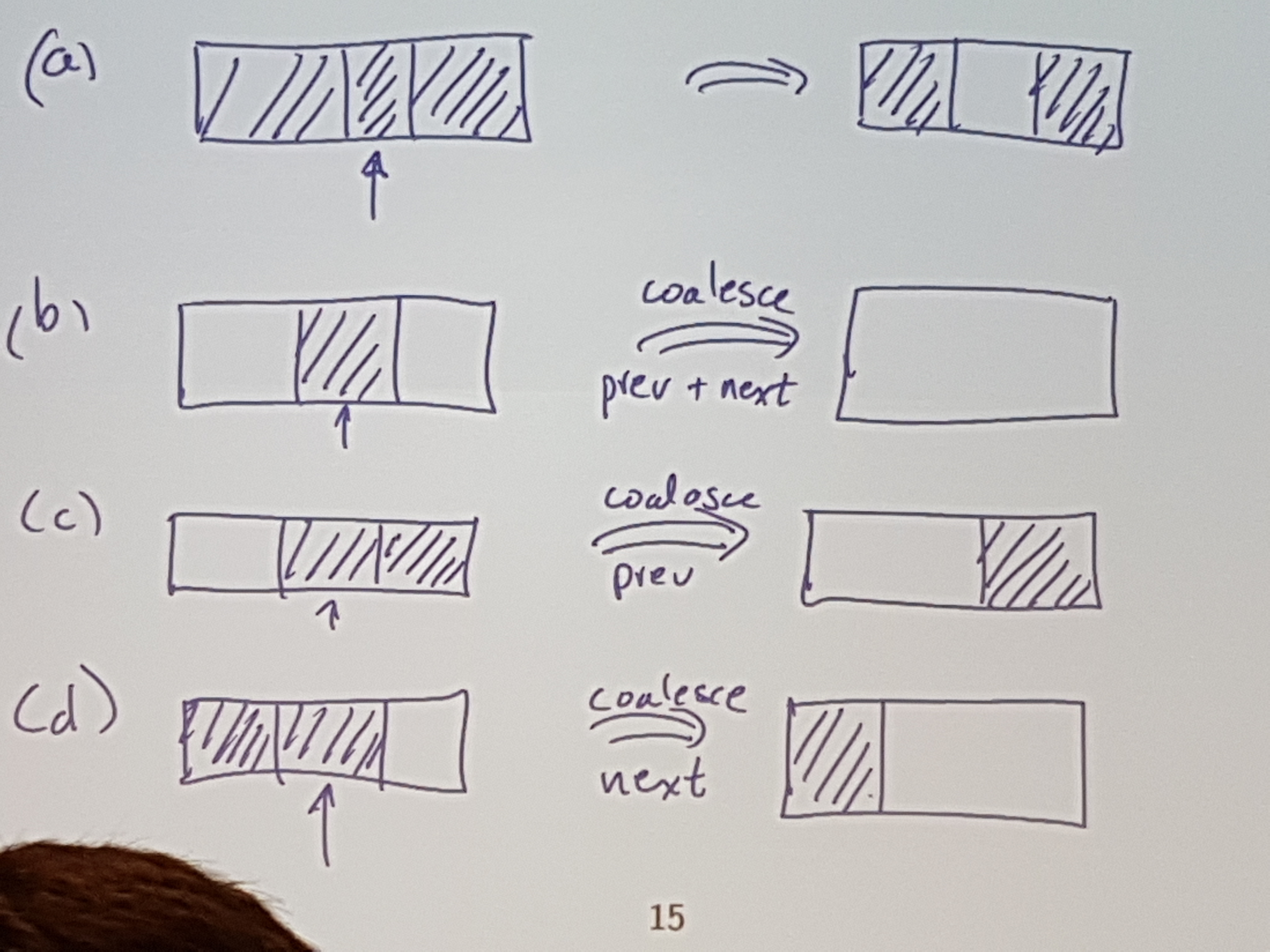## Buddy System : Initialize

The buddy system is what alloc.merl uses.

Each block has two metadata features:
- Size: Number of words in the block
- Code: Identification

Code length is inversely proportional to block size.

The code is used to identify my “buddy”

## Buddy System : AllocateAnd allocate the left one of those 128 blocks.

## Buddy system : Delete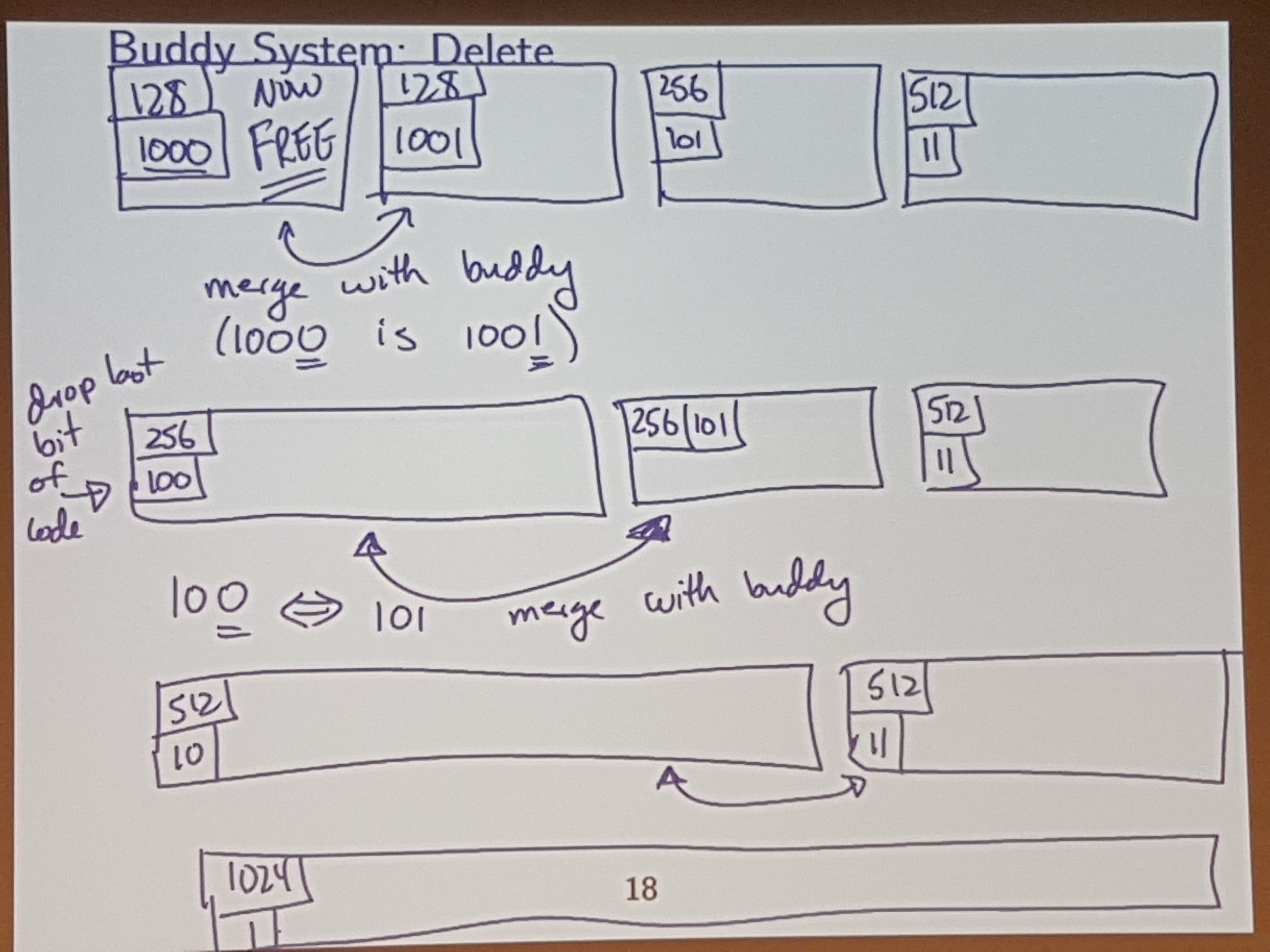## Deferred reclamation

Heuristic: if I allocate a block of size M, then there is a goodchance that later I may ask for a block of the (same) size M.

Instead of coalescing as soon as you get the chance, just leave some adjacent blocks unmerged.

So, when do you coalesce? Once we reach an “OUT OF MEMORY” consition due to fragmentation (not actaully b/c we are out of memory)

## Using a dual core processor

If we have two cores, and we notice that we are about to hit one of these fragmentation conditions (say, around 80% full), we can dedicate 1 core to start coalescing the memory, while the other core continues to take requests for memory.

This is calles “Real-time garbage collection”

## An even better idea!

Copywrite Gord Cormack 2010:
“Use a non lame language” (heads up: C++ is lame)We can’t pull this off in C / C++ since with pointers, we have direct access to specific memory, and we can’t just shuffle around objects willy nilly.

## Comparison of copy / compaction

If we have a non-lame language, then one way to do this compaction is with copy / compaction.## Implicit reclamation

recall: implicit = programmer does not indicate “delete this”

Sometimes called garbage collection (Java does this)

In the 1950s, the way they did this was using something called “mark + sweep” => “find the garbage, and reclaim it”

Contemporarily:
From the 1980s and onwards, “copy + collect” => “find the good stuff and disregard the rest”

## Issues opened up

How do we actually determine what’s garbage vs used memory?

MATH 239 / CS 240
=> depth-first search - looking at memory / pointers as a directed graph
=> cycle finding algos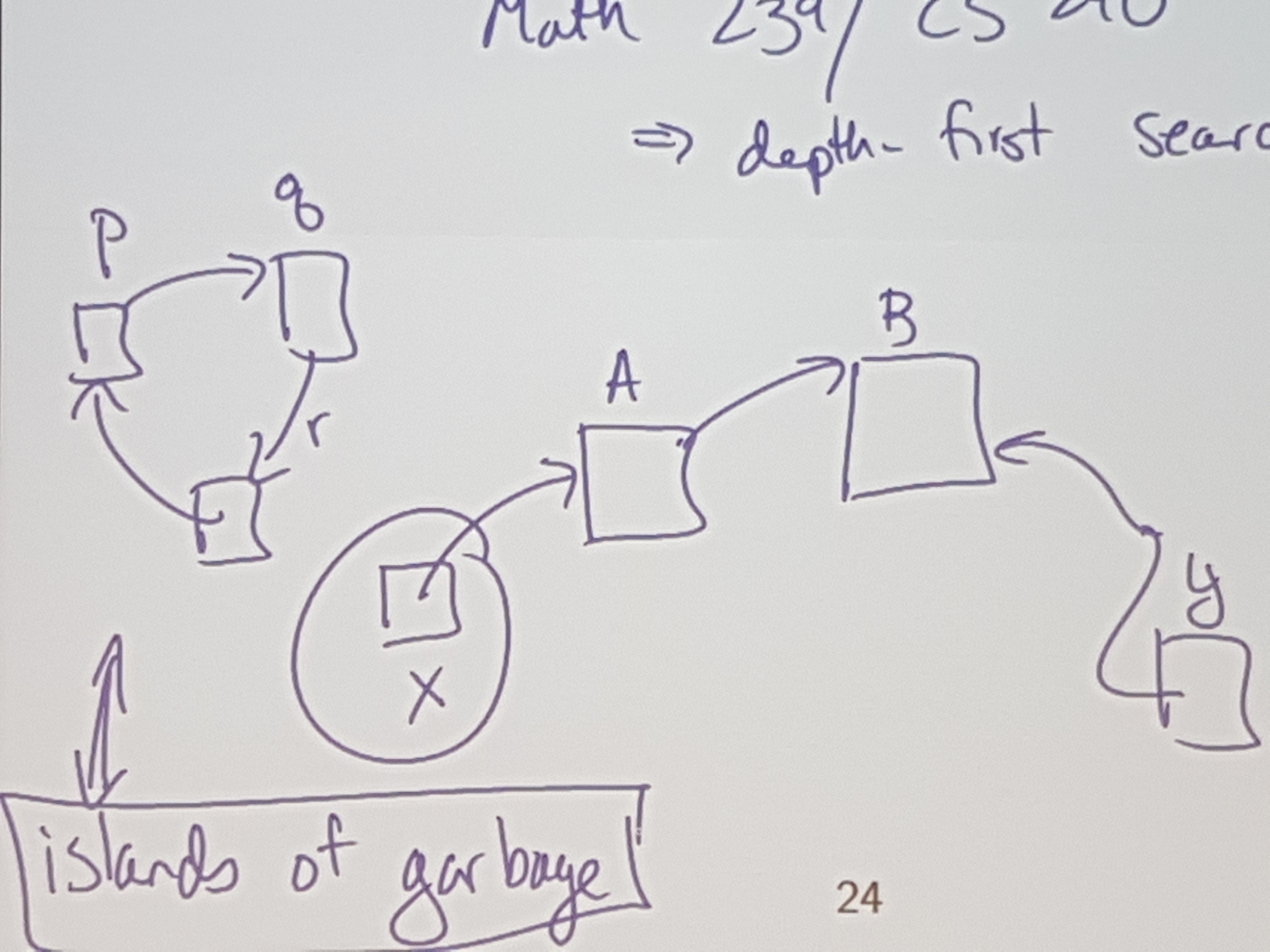Appel, a computer scientist, (wrote a textbook on compilers, and came up with copy / collect), said that stacks are obsolete.

He proposed that all pushes should be allocates, and all pops are marking memory as deallocated (using copy and correct of course)

Go is like C, but with safe pointers and garbage collection.
Go is a non-lame language.

# A few final words about CS241

## Mysteries Solved

This course is supposed to make sure that there are no mysteries left about how programs are compiled and run.

So, understand all the parts of the compiler (lexing, scanning, etc…), and how to run executables made of machine code (how memory works, how instructions get executes, etc..)

## Languages

Languages are more similar than different.
If statements tend to look similar across languages, even across lisp-like vs c-like languages.
In the end, a CPU is just sand, that can add, subtract, branch, etc… So no matter how much abstraction we throw onto that, in the end, an if-statement will always be an if statement.

## Breaking down problems

the journey of a throusand miles begins with one step - Confucius

A major takeaway: Don’t solve one big problem all at once. Break down problems into many smaller, manageable chunks.

## Abstraction

Computer science is the science of abstraction.

In CS, we only try to see the big picture.
We want to put things into a machine, and get stuff out of that machine as easily as possible.

The 1s and 0s are important, but int the end, they are only tools we use to get what we actually look for.

Takeaway: only focus on details that matter, WHEN they matter.

## Planning

Planning is always a good idea.

The first compiler was written for the langauge FORTRAN.
It took 18 person-years to make (on a team of 4)

Why did it take so long?
Well, they didn’t really have a plan going into it, and just tried to figure it out as they went.

## Specs REALLY matter

When all else fails, RTFM, and if there ain’t no manual, gg no re.